Journal DetailsFormat
Journal
eISSN
1891-5469
First Published
01 Jan 2010
Publication timeframe
1 time per year
Languages
EnglishOpen Access

# A short tutorial contribution to impedance and AC-electrokinetic characterization and manipulation of cells and media: Are electric methods more versatile than acoustic and laser methods?

###### Received: 07 Feb 2013
Journal DetailsFormat
Journal
eISSN
1891-5469
First Published
01 Jan 2010
Publication timeframe
1 time per year
Languages
English

Lab-on-chip systems (LOCs) can be used as in vitro systems for cell culture or manipulation in order to analyze or monitor physiological cell parameters. LOCs may combine microfluidic structures with integrated elements such as piezo-transducers, optical tweezers or electrodes for AC-electrokinetic cell and media manipulations. The wide frequency band (<1 kHz to >1 GHz) usable for AC-electrokinetic manipulation and characterization permits avoiding electrochemical electrode processes, undesired cell damage, and provides a choice between different polarization effects that permit a high electric contrast between the cells and the external medium as well as the differentiation between cellular subpopulations according to a variety of parameters. It has been shown that structural polarization effects do not only determine the impedance of cell suspensions and the force effects in AC-electrokinetics but can also be used for the manipulation of media with inhomogeneous temperature distributions. This manuscript considers the interrelations of the impedance of suspensions of cells and AC-electrokinetic single cell effects, such as electroorientation, electrodeformation, dielectrophoresis, electrorotation, and travelling wave (TW) dielectrophoresis. Unified models have allowed us to derive new characteristic equations for the impedance of a suspension of spherical cells, TW dielectrophoresis, and TW pumping. A critical review of the working principles of electro-osmotic, TW and electrothermal micropumps shows the superiority of the electrothermal pumps. Finally, examples are shown for LOC elements that can be produced as metallic structures on glass chips, which may form the bottom plate for self-sealing microfluidic systems. The structures can be used for cell characterization and manipulation but also to realize micropumps or sensors for pH, metabolites, cell-adhesion, etc.

#### Keywords

Introduction

While micro total analysis systems (μTAS) [Daridon et al. 2001, Kovarik et al. 2012] were originally developed for chemical analysis, lab-on-chip systems (LOCs) [Becker et al. 1995, Dürr et al. 2003, El-Ali et al. 2006, Fuhr et al. 1997, Goater et al. 1997, Wolf et al. 2006] were developed to monitor physiological cell parameters, cell manipulation and sorting, or for use as micro-bioreactors. Nowadays, a distinction between the two types of microsystems seems somewhat obsolete. With integrated sensors, the microsystems can be used for non-invasive, on-line monitoring of a cell’s physiological state and the effects of specific compounds [Bousse et al. 1994, Bousse and Parce 1994, Buehler et al. 2011] while neuro-chips can detect the action-potential patterns of electrically active neuronal networks [Gross et al. 1995, Haia and Spira 2012, Koester et al. 2010a]. Automated patch-clamp systems combine microfluidic structures to handle suspended single cells with patch electrodes for electrophysiological investigations [Koester et al. 2010b, Dunlop et al. 2008, Py et al. 2011]. The development of cell-based in vitro systems aims at reducing animal testing and costs in medical diagnostics or drug development. In these systems, microfluidic structures can be combined with integrated manipulation and sensor elements for cell or particle alignment, orientation, sorting, trapping, deformation or cell lysis and analysis [Barat et al. 2012, Becker et al. 1995, Dürr et al. 2003, Fiedler et al. 1998, Fuhr and Reichle 2000, Goater et al. 1997, Laurell et al. 2007, Nilsson et al. 2009, Sun and Morgan 2010, Schnelle et al. 2000].

Three different force fields are commonly used for contactless manipulation of biological particles or cells in microchips. All three are based on differences in the effective physical properties of the objects and their surrounding media. While optical traps (laser tweezers) exploit differences in the refractive indices (corresponding to permittivity differences at light frequencies for nonmagnetic objects) and are usually applied to trap single objects [Fuhr and Reichle 2000], acousto-fluidic cell manipulation methods have been elaborated for the complex handling of a multitude of objects including cells [Barat et al. 2012, Nilsson et al. 2009]. The technique is based on differences in the viscoelastic properties of the objects and the suspension medium. New setups allow for alignment, orientation, separation, sorting, and trapping [Guck et al. 2005, Laurell et al. 2007, Nilsson et al. 2009, Oberti et al. 2010]. Meanwhile, the performance of acoustofluidics [Glynne-Jones and Hill 2013] is comparable to that of earlier AC-electrokinetic techniques [Dürr et al. 2003, Müller et al. 1999, Schnelle et al. 2000].

In contrast to DC-electrokinetic techniques, such as electrophoresis and electro-osmosis, which are based on the interaction of permanent charges with the applied electric field, AC-electrokinetic techniques are based on the interaction of induced charges with the applied electric field. Accordingly, the induced force effects are based on differences in the effective electric polarizability of the objects and the suspension medium. Because AC-electrokinetics covers a larger frequency range than optical and acoustic methods and spans many frequency decades (<1 kHz to >1 GHz), AC-electrokinetics is more flexible in the choice of polarization effect to be exploited in the generation of the manipulation forces [Jones 1995, Hölzel 1997]. For a historical overview please see Gimsa et al.  and Jones .

Generally, the forces generated increase with the contrast between the objects and their surrounding media, i.e. the differences in the effective refractive indices, the viscoelasticities and the electric polarizabilities in optical, acoustic and AC-electrokinetic manipulation, respectively. For manipulation, an optimal gradient with respect to the object size and at an overall low energy absorption in and around the object is desirable. Different methods exist to increase the contrast for the three force fields, i.e. choosing an optimal field frequency/wavelength, temperature adjustment, staining, adjustment of the external medium properties, e.g. by changing viscosity and density in acoustic manipulation or in lowering the medium conductivity in AC electrokinetics.

Even though absorption will mainly result in temperature dissipation in all three techniques, the primary mechanisms of energy absorption are very different, ranging from direct acousto-mechanical vibrations to the absorption of single light quanta. Nevertheless, to the best of our knowledge the energy absorption in and around the objects, i.e. the actual load for the biological cells has not been compared for the three methods (for AC fields see Wachner et al. , Retelj et al. , Simeonova et al. ).

Of course, it is advantageous if the property contrasts can be directly (label-free) exploited for the (physiological) characterization and/or handling of the object, such as is the case with the elastic properties of cancerous cells [Guck et al. 2005], the cell-membrane conductivity [Gimsa et al. 1989] or the abundance of membrane systems in the cells [Gimsa et al. 1991]. It would also be interesting to combine non-interfering force fields for certain manipulation purposes or to consider the cross talk of the induced effects [Barat et al. 2012, Fuhr and Reichle 2000, Schnelle et al. 2000].

Because AC-electrokinetics and electric impedance measurements use the same frequency range, both methods may (in principle) detect the same properties, e.g. cell proliferation in suspensions. Nevertheless, with adherent cells, only the impedance can be detected, e.g. from interdigitated electrode structures (IDES), preferably in the low kHz range [Ehret et al. 1997, Koester et al. 2010a].

This short review focuses on the various AC-electrokinetic effects that can be exploited in LOCs [Gimsa 2001, Jones 1995, Shih et al. 2013]. The frequency dependence of AC-electrokinetic effects such as electroorientation (EO), electrodeformation (ED), translation (dielectrophoresis; DP), aggregation or electrorotation (ER) can be used to characterize suspended single cells [Gimsa 1999, Gimsa et al. 1991, Griffin 1970]. These effects can also be exploited in the manipulation of cells for sorting, collecting and positioning in LOCs [Fuhr et al. 1997, Schnelle et al. 2000]. AC-electrokinetic effects arise from the interaction of the induced polarization of the objects with the inducing external field. Unlike impedance, AC-electrokinetic characterization requires a sufficiently high field strength (>1000 V/m) and the (microscopic) observability of the induced movements. Its advantage is a higher parameter resolution and the discrimination against electrode effects. Whereas impedance methods are integrative, AC-electrokinetic methods are differential, i.e. impedance determines the integral polarizability of suspension medium and the objects, while AC-electrokinetics is based on the difference in the effective polarizabilities of medium and object. Nevertheless, in both approaches, analytical descriptions are based on the same geometrical models, e.g. multi-shell ellipsoidal cell models.

While the observed effect depends on the field quality, the frequency dependence of the dipole moment induced in the object generates particular frequency spectra. These relations can be easily explained for DP and ER: while objects subject to DP are translated in a linear inhomogeneous field, they rotate in a circular homogeneous field in ER. The DP force and ER torque are proportional to the in-phase (mathematically: the real) and out-of-phase (mathematically: the imaginary) component of the induced dipole moment, respectively [Gimsa and Wachner 1998, Jones 1995]. Combining DP and ER spectra is ideal for the dielectric characterization of single objects, because the methods permit a method-based separate access to the real and imaginary components of the induced dipole moment. Nevertheless, the AC-electrokinetic effects are not independent of each another, and combined force spectra must be expected, e.g. broken DP and ER spectra with ranges separated by the reorientation of non-spherical objects. Such spectra can be observed in combined measurements of the EO, DP and ER spectra of ellipsoidal chicken red blood cells in isotonic media of different conductivities. An ellipsoidal single-shell model is the simplest possible approach for the explanation of such combined effects [Gimsa 2001, Yang and Lei 2007].

Interestingly, AC-electrokinetic forces can also be observed in the bulk of fluids when dielectric property gradients are introduced by local heating. In electrothermal traveling wave (TW) micropumps (ETTWμPs) [Fuhr et al. 1992 and 1994, Gimsa et al. 1997, Morgan et al. 2001, Perch-Nielsen et al. 2004, Liu et al. 2014] and electro-thermal micropumps (ETμPs) [Stubbe et al. 2013], the interaction of the external AC field with smeared polarization charges of the medium induces medium pumping.

Many of the cell manipulation or pumping elements can be produced as platinum or transparent indium-tin-oxide structures on glass chips, which may form the bottom plate of measuring chambers or self-sealing microfluidic systems.

Please note, that electric force effects on suspended objects or media can also be induced by the interaction of DC or AC fields with electric double layer (see: DC electrokinetics) or dipole charges (see: Debye effects). When an external AC field deforms the equilibrium distributions of such charges, objects or media are polarized. This polarization, as well as its frequency-dependent α-, β-, γ-, and δ-dispersions, may generate AC electrokinetic effects. Nevertheless, this paper focuses on AC field-induced polarizations at structural interfaces in inhomogeneous media (Maxwell-Wagner (i.e. β) polarizations and their dispersions). For a summary see e.g. Foster and Schwan , Gimsa and Wachner , Schwan et al. .

Polarization of objects

The AC fields induce polarization charges at the structural interfaces inside inhomogeneous media (Maxwell-Wagner polarization). In suspensions or emulsions of micro- and nano-scale objects such as particles, oil droplets, air bubbles and biological cells, these structures are introduced by medium boundaries, e.g. biological membranes. Nevertheless, local heating may also introduce "smeared interfaces" into homogeneous bulk media, e.g. aqueous electrolytes. AC-electrokinetic effects are based on the forces generated by the interaction of these polarization charges with the inducing field. The observed effect depends on the temporal and spatial field properties. For objects, EO, ED, DP, ER, field-trapping/caging and travelling wave dielectrophoresis (TWDP) are well investigated. The frequency dependence of the dipole moment induced in the objects or volumes generates particular frequency spectra that provide information on the dielectric properties of the objects. Nevertheless, heated micro and nano volumes of a medium experience analogous forces, which can be used e.g. for pumping and mixing.

For biological cells, the general dielectric structure is introduced by two media of complementary electrical properties. While biological membranes have relative permittivities below 10 and specific conductivities in the range of 10-6 S/m, aqueous solutions (cytoplasm, nucleoplasm and interstitial solution) have values of approximately 70 and 1 S/m, for their respective properties. Thus, from a physical point of view, the lipid and aqueous phases can qualitatively be represented by air (or vacuum) and water, respectively. The simple block-model in Figure 1 consists of air and water blocks confined by electrodes. The electrode charges will influence charges in water but not in air. These interfacial charges are carried by ions (according to conductivity differences) and dipoles (according to the permittivity differences).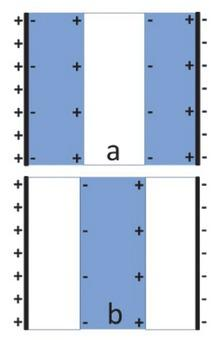Two complementary models consisting of blocks of air and water representing the membranous and aqueous media in cellular systems. a) Air block confined by two water blocks. b) Water block confined by air blocks. The external electrode charges influence charges in water but not in air. Induced charges are drawn for DC or AC for the half-wave during which the left electrode is positively charged.

A geometrical transition into spherical objects, i.e. a water droplet and an air bubble immersed in air and water, respectively, will not alter the charge distributions qualitatively.

Figure 2 shows the two complementary cases of the effective polarization of spherical objects that are less or more polarizable than their surrounding medium. Please note that their size allows for an electroquasistatic description of the polarization of micro-objects such as cells up to above 1 GHz. Figure 3 presents the single-shell model of a biological cell. For low external conductivity, biological cells may qualitatively behave like air bubbles (below membrane dispersion) or water droplets (above membrane dispersion) depending on the field frequency. While the cytoplasm is almost field-free at low frequencies, the membrane experiences extremely high field around its poles oriented in field direction (in a qualitative view the voltage dropping above the cell drops above its membrane).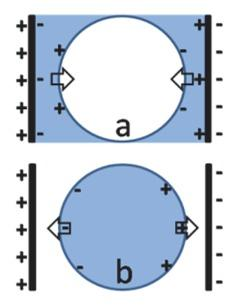Complementary polarization and force effects in air-water models. Induced charges are located in the medium with the higher polarizability. The interaction of these charges with the electrode charges, i.e. the external field, leads to a compression of the air bubble (a) and an elongation of the water droplet (b).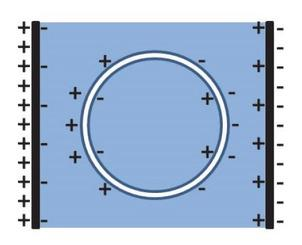Single-shell cell model obtained by introducing the water droplet (Figure 2b) into a slightly larger air bubble (Figure 2a), leaving an air film (i.e. a "biological membrane") around the droplet’s surface. It was assumed that the external bulk medium has a lower conductivity than the internal bulk while both bulk media are isotonic.

Generally, AC-electrokinetic effects are modelled using the induced dipole moment of the objects. The dipole moment is proportional to the difference of the integrals over the effective local fields in the volume of the object and the undisturbed external field in the same volume in the absence of the object. The second integral is frequency-independent for constant external field strength. In the biological cell (Figure 3), the volume integral is dominated by the membrane field at low frequencies. At high frequencies, the membrane is capacitively bridged, the membrane-field contributions fade and the volume integral is dominated by the cytoplasmic field. Please note that the orientations of the dipole moments are antiparallel in the two cases.

According to physical principles, forces are generated by potential differences, e.g. the difference between the potentials at the site of the pole in the presence and absence of the object (Figure 4). Please note that the effective field in confocally shelled objects polarized in a homogeneous external field is constant and corresponds to that of Maxwell's equivalent body.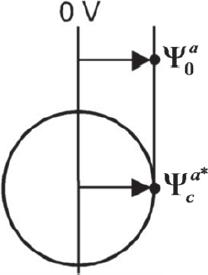The (horizontally oriented) external field (component) Ea induces the potentials Ψ0a=zEa  and  Ψca*$\Psi _{0}^{a}=z{{E}_{a}}\,\,and\,\,\Psi _{c}^{a*}$in the absence (subscript 0) and the presence (subscript c) of an ellipsoidal (cellular) object at the site of the pole of its semiaxis a. Ψca*$\Psi _{c}^{a*}$is highest for a vacuum object with a polarizability comparable to that of a membrane covered cell at low frequency. For example, a vacuum sphere attracts the equipotential plane that is located at the "influential radius" ainf distance of 3a/ 2 from the symmetry plane, which can be assumed to be at 0 V. While the maximum of Ψca*=ainfEa$\Psi _{c}^{a*}={{a}_{\inf }}{{E}_{a}}$is determined by the influential radius, i.e. the geometry of the object, its actual value is determined by the effective electric properties of object and suspension medium.

Our influential-radius concept allows for the separation of the geometric and electric problems and a simple description of the induced dipole moment, in addition to the transmembrane potential [Gimsa and Wachner 2001]. The influential radius describes the dependence of the maximum pole potential to the shape of an ellipsoidal object. Generally, the induced complex dipole moment along semiaxis a for an object of volume V = 4π abc / 3 is:

$ma*=εeε0 VfCMa*Ea$$$m_{a}^{*}={{\varepsilon }_{e}}{{\varepsilon }_{0}}\,Vf_{CM}^{a*}{{E}_{a}}$$

with $εeε0,fCMa*=fCMaℜ+fCMaJ and Ea$${{\varepsilon }_{e}}{{\varepsilon }_{0}},f_{CM}^{a*}=f_{CM}^{a\Re }+f_{CM}^{a\mathfrak{J}}\,\text{and}\,{{E}_{a}}$being the permittivity of the external medium, the complex Clausius-Mossotti factor consisting of its real and imaginary part and the external field component along semiaxis a. Complex terms are marked by asterisks.

$fCMa*$$f_{CM}^{a*}$is given by the normalized potential difference at the site of pole a in the presence and absence of the ellipsoidal object (Figure 4):

$fCMa*=1na(Ψ0a−Ψca*Ψ0a)$$$f_{CM}^{a*}=\frac{1}{{{n}_{a}}}\left( \frac{\Psi _{0}^{a}-\Psi _{c}^{a*}}{\Psi _{0}^{a}} \right)$$

with na being the depolarizing factor along semiaxis a [Gimsa 2001]. Please note that na + nb + nc =1 for ellipsoidal objects, i.e. na = nb = nc =1/ 3 for spherical objects. The distances from the object’s respective symmetry planes to those equipotential planes that are just touching the respective poles of a vacuum object with the shape of the actual object are along each semiaxis of an ellipsoidal object ainf , binf , cinf . Along semiaxis a the influential radius is:

$ainf=11−naa$$${{a}_{\inf }}=\frac{1}{1-{{n}_{a}}}a$$

While the three influential radii provide the solution for the geometric problem, the electric problem for a single shell ellipsoid can be solved, assuming a series circuit of three resistor-capacitor (RC) pairs for the internal, membrane and external media along each semiaxis. The length of the three external elements (e.g. $Zea*$$Z_{e}^{a*}$along semiaxis a) must be such that it ensures the maximal possible potentials at the poles.

There are at least two arguments for the correctness of a description by three stacked "finite" elements (RC pairs) along each semiaxis:

For symmetry reasons, field lines must exist that are oriented along the semiaxes. For a current along these field lines, the voltage drop relations are correctly described by chains of RC pairs with infinitely small but equal cross sections (equation (5)).

In analogy to Maxwell's equivalent-sphere notion, the fields in the ellipsoidal internal homogeneous bulk (cytoplasm) and in the equivalent body of the whole single shell object are constant. These fields (or more general: field components) are oriented in parallel to the inducing external field (or field components). Accordingly, the internal bulk and the membrane-field components at the poles are oriented along their respective semiaxis and the voltage drops over the membrane correspond to the induced transmembrane potentials, i.e. the differences of the potentials at the poles of the internal bulk object and the whole object.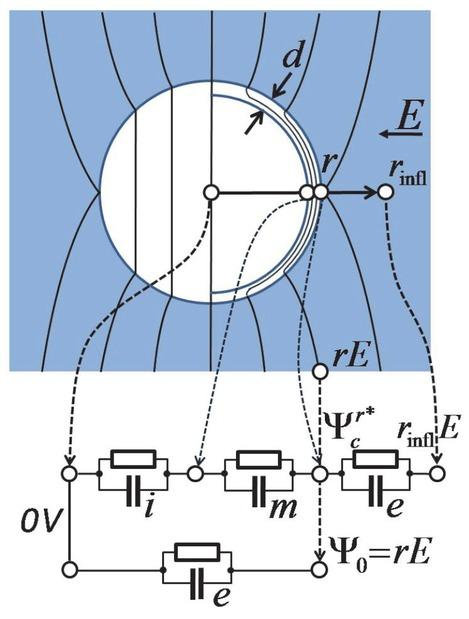Equipotential plane distribution in and around a spherical single-shell model of radius r . The external distributions are identical for Maxwell's equivalent body (sketched for the left hemisphere) and the membrane-covered object (sketched for the right hemisphere). Important points in the model are marked by circles. The potentials at these points can be obtained from the RC-model at the bottom, which permits calculation of the induced dipole moment and transmembrane potential. From the existence of Maxwell's equivalent body, it follows that electric measurements (detecting the induced dipole moment) do not allow one to distinguish whether frequency-dependent object properties stem from internal structures or from the frequency-dependent properties of the media of which the object is composed.

The impedances Z* of the three RC pairs along a semiaxis can be calculated from the geometries of prismatic elements given by their lengths l , i.e. length of the semiaxis ( l = a), membrane thickness ( l = d), and ( l = ainfa), as well as a constant cross-sectional area A:

$Z*=1σ+jωεε0lA$$${{Z}^{*}}=\frac{1}{\sigma +j\omega \varepsilon {{\varepsilon }_{0}}}\frac{l}{A}$$

with j= (1)0.5and ω = 2π f , where σ , ε , ε0, and f stand for the specific conductivity and relative permittivity of the considered medium, the permittivity of vacuum and the field frequency. From the voltage-divider chains, the potentials at the semiaxes poles can be obtained. Along semiaxis a we obtain:

$Ψca*=Zia*+Zma*Zia*+Zma*+Zea*ainfaΨ0a$$$\Psi _{c}^{a*}=\frac{Z_{i}^{a*}+Z_{m}^{a*}}{Z_{i}^{a*}+Z_{m}^{a*}+Z_{e}^{a*}}\frac{{{a}_{\inf }}}{a}\Psi _{0}^{a}$$

with $Ψ0aa=E0a$$\frac{\Psi _{0}^{a}}{a}=E_{0}^{a}$being the external field or field component in the direction of semiaxis a. The maximum possible potential at the pole is $ainfE0a,$${{a}_{\inf }}E_{0}^{a},$which is reached at low enough frequencies for an infinitely high membrane impedance $Zma*$$Z_{m}^{a*}$. Analogously, the transmembrane potential $ΔΨma*$$\Delta \Psi _{m}^{a*}$at the pole of semiaxis a is:

$ΔΨma*=Zma*Zia*+Zma*+Zea*ainfE0a$$$\Delta \Psi _{m}^{a*}=\frac{Z_{m}^{a*}}{Z_{i}^{a*}+Z_{m}^{a*}+Z_{e}^{a*}}{{a}_{\inf }}E_{0}^{a}$$

The RC models obtained for the induced dipole moment and the transmembrane potential can easily be simplified for the limiting DC and very high-frequency cases, and for certain frequency bands or dispersion processes [Gimsa and Wachner 1999]. For ns pulses see Retelj et al.  or Schoenbach et al. .

The behavior of the cell model in the dispersion range of membrane polarization is of special interest. For a qualitative consideration, the permittivities of the internal and external bulk media, as well as the membrane conductivity, can be neglected. For simplicity, a spherical cell of radius a = r and ainf = rinf = 3r /2 is considered. The three finite elements described by equation (4) are reduced to:

$Zi=rσiA,Zm*=1jωεmε0dA=1jωCmspecA, and Ze=r2σeA$\begin{align}& \,\,\,\,\,\,\,\,\,\,\,\,\,\,\,\,\,\,\,\,\,\,{{Z}_{i}}=\frac{r}{{{\sigma }_{i}}A}, \\ & Z_{m}^{*}=\frac{1}{j\omega {{\varepsilon }_{m}}{{\varepsilon }_{0}}}\frac{d}{A}=\frac{1}{j\omega C_{m}^{spec}A},\,\,\text{and} \\ & \,\,\,\,\,\,\,\,\,\,\,\,\,\,\,\,\,\,\,\,\,\,{{\text{Z}}_{e}}=\frac{r}{2{{\sigma }_{e}}A} \\ \end{align}

with d , ε m, and $Cmspec=Cm/A$$C_{m}^{spec}={{{C}_{m}}}/{A}\;$being the thickness, relative permittivity and area specific capacitance of the membrane. In the following, the induced membrane potential is considered as an example to demonstrate the simplicity of the influential radius ansatz. Using equations (7) and expanding the fraction by $1/Zm*$${1}/{Z_{m}^{*}}\;$equation (6) reads:

$ΔΨm*=rinf1+Zi+ZeZm*E0rinf1+jω(rσi+r2σe)CmspecE0$$$\Delta \Psi _{m}^{*}=\frac{{{r}_{\inf }}}{1+\frac{{{Z}_{i}}+{{Z}_{e}}}{Z_{m}^{*}}}{{E}_{0}}\frac{{{r}_{\inf }}}{1+j\omega \left( \frac{r}{{{\sigma }_{i}}}+\frac{r}{2{{\sigma }_{e}}} \right)C_{m}^{spec}}{{E}_{0}}$$

The characteristic frequency of membrane polarization is obtained from the RC-time constant of the membrane:

$fc1=ωc2π=12π(Zi+Ze)Cm=12π r Cmspec(1σi+12σe)$$${{f}_{c1}}=\frac{{{\omega }_{c}}}{2\pi }=\frac{1}{2\pi \left( {{Z}_{i}}+{{Z}_{e}} \right){{C}_{m}}}=\frac{1}{2\pi \,r\,C_{m}^{spec}\left( \frac{1}{{{\sigma }_{i}}}+\frac{1}{2{{\sigma }_{e}}} \right)}$$

Using equation (9), the absolute value of the induced transmembrane potential is obtained from equations (8) leading to the Schwan equation [Marszalek et al. 1990, Schwan 1983]:

$|ΔΨm*|=1.5rE0|11+jffc1|=1.5rE01+(ffc1)2$$$\left| \Delta \Psi _{m}^{*} \right|=1.5r{{E}_{0}}\left| \frac{1}{1+j\frac{f}{{{f}_{c1}}}} \right|=\frac{1.5r{{E}_{0}}}{\sqrt{1+{{\left( \frac{f}{{{f}_{c1}}} \right)}^{2}}}}$$

In spherical objects, the magnitude of the induced transmembrane potential depends on the cosine of the angle between a line from the considered membrane site through the center of the sphere and the semiaxis which is oriented in field direction. At its pole, the membrane field is at a maximum of (rinf /d)E0 , i.e. the external field is amplified by a factor of rinf /d corresponding to approximately 1000 for a standard cell geometry.

Using the influential radius notion to separate the geometric and electric problems, analogous derivations are possible for spheroidal or ellipsoidal objects with conductive membranes [Gimsa and Wachner 1999, Gimsa 2001].

AC electrokinetic effects observed with particles and cells

In AC electrokinetics, the observed effect depends on the field quality, i.e. the time-dependent distribution, orientation or inhomogeneity of the field. The frequency dependence of the induced dipole moment generates particular frequency spectra for the forces or torques. The sketches in Figures 6-8 summarize the effects observed in homogeneous (Figures 6, 7a), inhomogeneous (Figures 7b, c), rotating (Figure 8a), and TW fields (Figure 8b).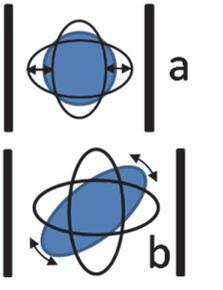(a) ED according to Figure 2. (b) For three-axial, ellipsoidal objects each axis may be oriented in parallel to the field. Orientation depends on field frequency, object and medium properties. The field electrodes as well as the possible (frequency-dependent) forces and torques are marked by bold dark lines and arrows, respectively.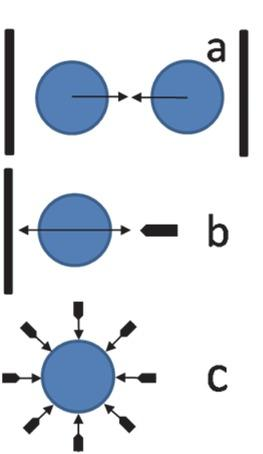(a) Evenly polarizable objects always attract one another (compare to charges in Figure 2) leading to the formation of pairs or pearl-chains. (b) Elongation and compression according to Figure 2a and b translate into positive or negative DP, i.e. movement of the object towards high and low field, respectively. (c) Strongly inhomogeneous fields can be used for the collection of submicro-objects or field caging.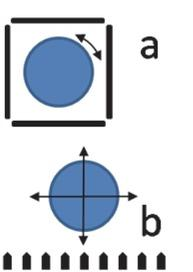(a) Rotating fields may induce a torque leading to object rotation in (increasing effective polarizability of object with frequency) or against (decreasing effective polarizability) the direction of field rotation. (b) This torque corresponds to the translational forces in TWDP. Lifting or attracting forces correspond to the DP forces in Figure 7b.

Equations describing the observed effects can be derived from the different kinds of interaction of the induced dipole moment and the inducing field. For simplicity, it is assumed that small objects are polarized in a homogeneous field, i.e. the characteristic distances of the field inhomogeneity are large in comparison to the object size. At field frequencies beyond the hydrodynamic time constants, the objects experience time-averaged effective forces and torques. Simplified equations can be derived within an orthonormal coordinate system of unit vectors i , j , k.

The ED force along the oriented semiaxis a (Figure 6a) is:

$〈Fa〉=ε0εeE2fCMaℜi$$$\left\langle {{F}_{a}} \right\rangle ={{\varepsilon }_{0}}{{\varepsilon }_{e}}{{E}^{2}}f_{CM}^{a\Re }i$$

the EO torque in linear polarized fields (Figure 6b) is:

$〈N〉=12εeε0 VE2(fCMbℜ−fCMcℜfCMcℜ−fCMaℜfCMaℜ−fCMbℜ)(ijk)$$$\left\langle N \right\rangle =\frac{1}{2}{{\varepsilon }_{\text{e}}}{{\varepsilon }_{0}}V{{E}^{2}}\left( \begin{matrix} f_{CM}^{b\Re } & - & f_{CM}^{c\Re } \\ f_{CM}^{c\Re } & - & f_{CM}^{a\Re } \\ f_{CM}^{a\Re } & - & f_{CM}^{b\Re } \\ \end{matrix} \right)\left( \begin{matrix} i \\ j \\ k \\ \end{matrix} \right)$$

(for EO in circular polarized fields, please see: [Gimsa 2001]). Evenly polarizable objects attract each other with:

$〈Fa〉=ε0εeVE2|fCMa|i$$$\left\langle {{F}_{a}} \right\rangle ={{\varepsilon }_{0}}{{\varepsilon }_{e}}V{{E}^{2}}\left| f_{CM}^{a} \right|i$$

over distances larger than the object size, where $|fCMa|=|fCMaℜ|2+|fCMaℑ|2$$\left| f_{CM}^{a} \right|=\sqrt{{{\left| f_{CM}^{a\Re } \right|}^{2}}+{{\left| f_{CM}^{a\Im } \right|}^{2}}}$. For DP (Figure 7b) and AC-field trapping (Figure 7c) or the vertical attraction and lifting in TWDP (Figure 8b) the force is:

$〈Fa〉=γ2ε0εefCMaℜE2i$$$\left\langle {{F}_{a}} \right\rangle =\frac{\gamma }{2}{{\varepsilon }_{0}}{{\varepsilon }_{e}}f_{CM}^{a\Re }{{E}^{2}}i$$

with γ standing for the site-dependent degree of field inhomogeneity.

Objects trapped by positive DP are consequently attracted to the areas of highest field strength, i.e. to electrode sites of high curvature. Even though electrode contact may be acceptable for the trapping of multitudes of small objects with passivated electrodes or at frequencies high enough to avoid electrode processes, negative DP (Figure 7c) is safer [Fuhr and Reichle 2000, Gimsa 1999, Grom et al. 2006, Schnelle et al. 2000]. Please note that optical traps exploit 'positive DP', i.e. a polarizability (permittivity/refractive index) of the (nonmagnetic, biological) objects that is higher than that of the surrounding medium at light frequencies. This is possible because field maxima can be generated at a distance from the optical 'antennas' (i.e. lenses, etc.) due to the different wave length - antenna size ratios at radio and optical frequencies.

Usually, 4-electrode chambers driven by 90°-phase-shifted signals are used to generate rotating electric fields (Figure 8a). The induced dipole moment rotates with the frequency of the inducing field. Nevertheless, in case of dispersion of the induced dipole moment at a given frequency, the dipole moment possesses a phase-shift with respect to the external field vector. Their interaction generates a permanent torque N c around semiaxis c:

$〈Nc〉=ε0εeVE2fCMaℜ+fCMbℜ2k$$$\left\langle {{N}_{c}} \right\rangle ={{\varepsilon }_{0}}{{\varepsilon }_{e}}V{{E}^{2}}\frac{f_{CM}^{a\Re }+f_{CM}^{\mathfrak{b}\Re }}{2}k$$

The torque is highest when the frequency of the exciting field corresponds to the relaxation time of a polarization process. The reason is that only the out-of-phase component of the induced dipole moment contributes to torque generation (cross-product of field and induced dipole moment). Accordingly, dispersion processes are a prerequisite of ER. To the best of our knowledge no attempts were published to detect an equivalent torque generated by circularly polarized light in dispersion ranges of the refractive index.

TWDP (Figure 8b) can be considered as a linearization of ER [Hagedorn et al. 1992, Hughes et al. 1996]. The traveling effect generates a field gradient in the traveling direction of the field. For an object oriented with semiaxis a in the traveling direction of the field and an object-related field distortion that is small in comparison to the electrode-period length (ainf << nel x), the TWDP force is:

$〈Fa〉=πε0εeVnelxE2fCMaℑi$$$\left\langle {{F}_{a}} \right\rangle =\frac{\pi {{\varepsilon }_{0}}{{\varepsilon }_{e}}V}{{{n}_{el}}\text{x}}{{E}^{2}}f_{CM}^{a\Im }i$$

with x and nel standing for the electrode distance and the number of electrodes for a full field period, e.g. four electrodes driven by 90°-progressively phase-shifted signals.

Please note that in the considerations above, the field magnitudes and time-dependent orientations in equations (7) to (12) depend on how the external field is defined. Because the effects are not independent of each other, combined force spectra are observed, e.g. the reorientation of non-spherical objects in linear (inhomogeneous) DP, rotating ER, and TW fields, respectively (for details see: Gimsa , Goater et al. , and Becker et al. ).

Impedance of suspensions

The influential-radius concept can easily be extended to describe the impedance of suspensions of three-axial single-shell cells or homogeneous particles using the mixing equations of Maxwell and Wagner [Pauly and Schwan 1959, Asami et al. 1980]. For a random orientation, each of the three different Clausius-Mossotti factors contributes by one third (this is equivalent to the notion that each of the three semiaxes of all particles evenly contributes to the impedance of a population of even ellipsoidal particles):

$3(σS*−σe*)σS*+2σe*=p3∑k=a,b,cfCMk*$$$\frac{3\left( \sigma _{S}^{*}-\sigma _{e}^{*} \right)}{\sigma _{S}^{*}+2\sigma _{e}^{*}}=\frac{p}{3}\sum\limits_{k=a,b,c}{f_{CM}^{k*}}$$

where σ * and p refer to complex specific conductivities and the volume portion of the suspended objects, whereas the indices S and e refer to the whole suspension as well as the external medium. Please note that the factor 3 in the left-hand term stems from the definition of the Clausius-Mossotti factor [Asami et al. 1980]. Accordingly, for objects oriented with semiaxis a in the external field direction we obtain:

$3(σS*−σe*)σS*+2σe*=pfCMa*$$$\frac{3\left( \sigma _{S}^{*}-\sigma _{e}^{*} \right)}{\sigma _{S}^{*}+2\sigma _{e}^{*}}=pf_{CM}^{a*}$$

This expression is generally identical to that given by Foster and Schwan  (equation 44a on page 44).

Synchronous reorientations of a population of suspended particles should be detectable as impedance transitions. For example, such reorientation can be induced by EO in DC, AC, optical, acoustic or hydrodynamic fields. The impedance of single, oriented cells can be detected in microfluidic devices [Sun and Morgan 2010].

Finally, the combination of equation (17) with equations (2) and (5) permits a simple theoretical description and the determination of cell parameters from experimental spectra. For this, the impedance terms in equation (5), each of them describing an RC pair according to equation 4, provide an easy ansatz because they can directly be simplified at certain frequency ranges [Gimsa and Wachner 1999]. This allows for extending Schwan's RC model for a suspension of spherical cells [Pauly and Schwan 1959, Foster and Schwan 1996] to suspensions of ellipsoidal cells (manuscript in preparation).

Using equations (2), (5) and (7), from equation (18) for a spherical object ( a = r ) we directly obtain:

$σS*=σe2σe+σi+2p(σi−σe)ω r Cmspec−2jσeσi(1−p)2σe+σi−p(σi−σe)ω r Cmspec−jσeσi(p+2)$$$\sigma _{s}^{*}={{\sigma }_{\text{e}}}\frac{2{{\sigma }_{\text{e}}}+{{\sigma }_{i}}+2p\left( {{\sigma }_{i}}-{{\sigma }_{e}} \right)\omega rC_{m}^{\text{spec}}-2j{{\sigma }_{\text{e}}}{{\sigma }_{i}}\left( 1-p \right)}{2{{\sigma }_{\text{e}}}+{{\sigma }_{i}}+p\left( {{\sigma }_{i}}-{{\sigma }_{e}} \right)\omega rC_{m}^{\text{spec}}-j{{\sigma }_{\text{e}}}{{\sigma }_{i}}\left( p+2 \right)}$$

Assuming equal absolute values for the real and imaginary parts of $σS*$$\sigma _{S}^{*}$after some calculations, a new expression for the characteristic frequency of suspensions of spherical cells fc1IMP in the membrane dispersion frequency range can be derived:

$fc1IMP=12π Cmspecr(12σe+1σi)(2σe+(1−34p)σi2σe+σi)$$$f_{c1}^{IMP}=\frac{1}{2\pi \,C_{m}^{spec}r\left( \frac{1}{2{{\sigma }_{e}}}+\frac{1}{{{\sigma }_{i}}} \right)}\left( \frac{2{{\sigma }_{e}}+\left( 1-\frac{3}{4}p \right){{\sigma }_{i}}}{2{{\sigma }_{e}}+{{\sigma }_{i}}} \right)$$

Please note that equation (20) converges to equation (9) for infinitely low cell concentrations (p=0). Because the expression slightly differs from that given by Foster and Schwan  (equation 24) we checked that the limiting cases:

$fc1IMP=σe(4−3p)4πCmspecr for σi>>σe$$$f_{c1}^{IMP}=\frac{{{\sigma }_{e}}\left( 4-3p \right)}{4\pi C_{m}^{spec}r}\,\,\,\,\,\,\text{for}\,\,{{\sigma }_{i}}>>{{\sigma }_{e}}$$$fc1IMP=σe(4−p)12πCmspecr for σi=σe$$$f_{c1}^{IMP}=\frac{{{\sigma }_{e}}\left( 4-p \right)}{12\pi C_{m}^{spec}r}\,\,\,\,\,\,\text{for}\,\,\,{{\sigma }_{i}}={{\sigma }_{e}}$$$fc1IMP=σi2πCmspecr for σi<<σe$$$f_{c1}^{IMP}=\frac{{{\sigma }_{i}}}{2\pi C_{m}^{spec}r}\,\,\,\,\,\text{for}\,\,\,{{\sigma }_{i}}<<{{\sigma }_{e}}$$

correspond.

AC-electrokinetic spectra of single cells and the impedance of cell suspensions

In the following calculations, standard parameters of biological cells have been assumed in order to consider the induced transmembrane potential, the impedance of suspensions, as well as force and torque spectra, which are observed in AC electrokinetic experiments (Table 1).

Standard parameters of the spherical cell model.

ParameterValue
Cell radius (r)5 μm
Membrane thickness (d)8 nm
Conductivities
external (σe)0.1 S/m
membrane (σm)10-6 S/m corresponding to 125 S/m2
internal (σi)0.01σe to σi>>σe
Relative permittivities ε
external ( e)80
membrane (ε) mε9.04 corresponding to 0.01 F/m2
internal ( i)50
Volume fraction (p)≤10% (not for single cell calculations)
External field strength for calculation of transmembrane potential133.33 kV/m

The Clausius-Mossotti factor of a spherical cell of radius r with $fCMrℑ=fCMaℑ=fCMbℑ=fCMcℑ$$f_{CM}^{r\Im }=f_{CM}^{\mathfrak{a}\Im }=f_{CM}^{\mathfrak{b}\Im }=f_{CM}^{\mathfrak{c}\Im }$was calculated using equations (2) and (5) (Figure 9). Its real and imaginary parts are proportional to the spectra of the DP force (equation (14)) and ER torque (equation (15). The spectra are reduced to the membrane dispersion when the permittivities of the internal and external bulk media are neglected (dotted lines). Nevertheless, in experiments, a second dispersion is clearly observed in the DP and ER spectra. Its mechanism is based on the superseding of the distributions of field and polarization, which are dominated by the frequency-independent bulk conductivities by distributions according to the bulk permittivities which dominate at high frequencies. This effect is usually neglected for the induced transmembrane potential as obtained from equation (10). Its -3 dB frequency corresponds to the characteristic frequency of membrane dispersion, i.e. the first peak in the imaginary part of the Clausius-Mossotti factor.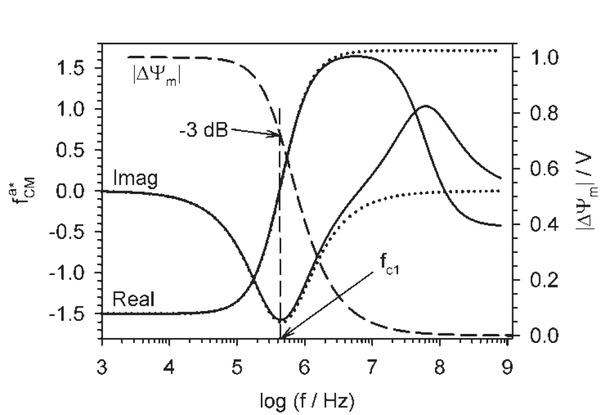Real and imaginary parts of the Clausius-Mossotti factor (full lines, left ordinate) as well as the induced transmembrane potential (dashed line, right ordinate) at the pole of a spherical cell model (Table 1). Dotted lines: spectra are reduced to the membrane dispersion when the permittivities of the bulk media are neglected. Vertical dashed line: -3 dB frequency of the absolute value of the induced transmembrane potential.

The specific impedance (compare to equation (4)) of a suspension of spherical cells was calculated by equation (18) for the parameters of Table 1 and p=0.1 (Figure 10, full lines).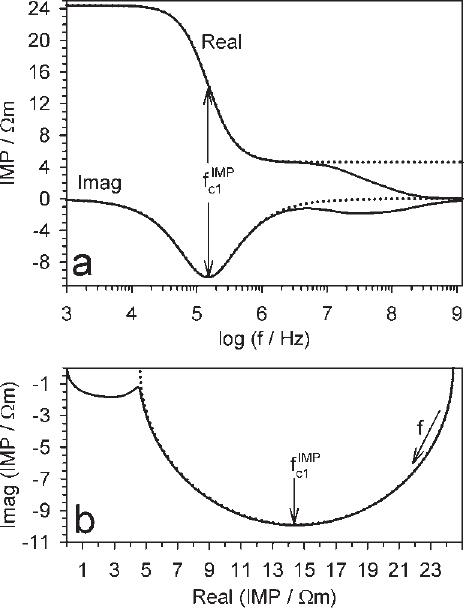Real and imaginary parts of the specific impedance (IMP=1/σS*)$\left( \text{IMP=}{1}/{\sigma _{S}^{*}}\; \right)$of a cell suspension over frequency (top), as well as in the complex plane (bottom) calculated for the parameters of Table 1 (full lines, equation (18)). The spectra are reduced to the membrane dispersion when the permittivities of the bulk media are neglected (dotted lines, equation (19)). The characteristic membrane-dispersion frequency of the suspension fc1IMP$f_{c1}^{IMP}$corresponds to the frequency of the peak in the imaginary part. In the complex plot, it is the frequency of the point obtained by dropping a perpendicular from the center of the semicircle.

Even though bulk-media dispersions are clearly observed in experiments with cell suspensions especially at low external conductivities, equation (18) has been reduced to the membrane dispersion. For this, the permittivities of the internal and external bulk media were neglected in the classical Laplace ansatz as well as in equation (5). Even though the derivation was simpler when started from the second approach, the same equation (19) was obtained in both cases (Figure 10, dotted lines). Besides the ease of simplifying an RC model, it is the ad hoc introduction of area-specific parameters for the membrane (equations (7)) that simplifies the derivation. An expression similar to equation (19) was not provided by Foster and Schwan .

From equation (19), an expression can be derived for the characteristic membrane-dispersion frequency $fc1IMP$$f_{c1}^{IMP}$(equation (20)). It was compared with the simplified equation 56b on page 46 in Foster and Schwan  assuming zero membrane conductance:

$fc1IMP=1+p2πCmspecr(12σe+1σi+p2(1σi−1σe))$$$f_{c1}^{IMP}=\frac{1+p}{2\pi C_{m}^{spec}r\left( \frac{1}{2{{\sigma }_{e}}}+\frac{1}{{{\sigma }_{i}}}+\frac{p}{2}\left( \frac{1}{{{\sigma }_{i}}}-\frac{1}{{{\sigma }_{e}}} \right) \right)}$$

The expressions are identical for cell-volume fractions of p=0, i.e. the characteristic frequency of a cell suspension $fc1IMP$$f_{c1}^{IMP}$(equation (20)) reduces to fc1 of the single cell (equation (9)). Consequently, equation (9) corresponds to equations (21) – (23) for p = 0.

Figure 11 compares different cases for the volume-fraction dependence of $fc1IMP$$f_{c1}^{IMP}$. At first, we checked that the $fc1IMP$$f_{c1}^{IMP}$plots of equation (18) correspond to the full expression of Foster and Schwan  (Figure 11, dotted lines). While $fc1IMP$$f_{c1}^{IMP}$slightly increases for increasing cell-volume fractions p in the simplified equation of Foster and Schwan  (full lines), our simplified equation (20) shows an improved correspondence to the full expression, with $fc1IMP$$f_{c1}^{IMP}$slightly decreasing with p.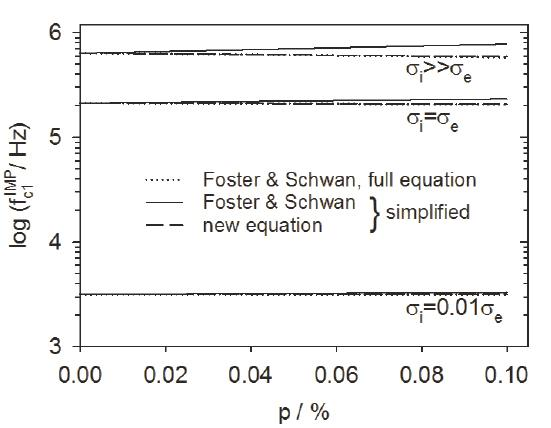Volume fraction dependencies of fc1IMP$f_{c1}^{IMP}$obtained from four different models for σi>>σe,   σi=σe,${{\sigma }_{i}}>>{{\sigma }_{e}},\,\,\,{{\sigma }_{i}}={{\sigma }_{e}},$and σi=0.001σe.${{\sigma }_{i}}=0.001{{\sigma }_{\text{e}}}.$Equation (18) corresponds to the complete model of Foster and Schwan  (dotted lines). While their simplified fc1IMP$f_{c1}^{IMP}$expression slightly increases with p (full lines), the new equation (20) predicts a moderate decrease (dashed lines), in accordance with the complete models. In all models, the points for p=0 are identical with fc1IMP=fc1$[f_{c1}^{IMP}={{f}_{c1}}$(equations 20-23).

In the literature, we did not find explicit experimental results on the cell-volume fraction dependence of $fc1IMP.$$f_{c1}^{IMP}.$Nevertheless, published data on blood cells reports an increase of the permittivity as well as a decrease of the conductivity for increasing volume fractions [Fricke 1953, Schwan et al. 1962]. The combination of these correlations suggests a decrease of $fc1IMP$$f_{c1}^{IMP}$for increasing volume fractions. Also, our own experiments showed a decrease of $fc1IMP$$f_{c1}^{IMP}$relative to fc1 for increasing fractions of human red blood cells, especially for p>0.1 (results not shown).

Despite the increasing capacities in the computing power of new computers, simplified equations will be useful for the qualitative understanding of the relations of detected parameters with cell and media properties. The equations also allow for fast data interpretation in screening applications or the on-line detection of cell properties, e.g. in batch cultures or microfluidic systems [Sun and Morgan 2010].

AC electrokinetic effects on media: pumping

Considerations of the structure-based, AC electrokinetic effects observed for objects are helpful for the intuitive explanation of analogous AC electrokinetic forces acting on the fluid media of ETTWμPs [Fuhr et al. 1992 and 1994, Perch-Nielsen et al. 2004] or ETμPs [Stubbe et al. 2013]. In electrothermal pumps, a temperature gradient alters the electric properties of the fluid media in certain regions of the pump medium. The actual temperature distribution in the pump media results from the interplay of internal and external (e.g. resistive or radiation) heating and heat dissipation across the channel walls and the metallic electrodes. Even though no defined structural interfaces are introduced by thermal gradients, forces evolve from the interaction of the field with the induced smeared charges which are qualitatively the same as at the interfaces of objects. The forces acting on the ion and dipole charges are transferred to water molecules, thereby inducing pumping. To induce a directed pump effect, thermally induced Prandtl convections should be avoided, in other words, thermal convections or the induced medium movement should not (significantly) change the temperature distribution [Stubbe et al. 2013]. For this, conductive heat dissipation should be high enough, i.e. the hydrodynamic cross-sections of the pump channels should be small and its surface-to-volume ratio high enough. For aqueous media around room temperature, these prerequisites are generally fulfilled at channel widths/heights below 400 μm. Please note that for plane electrode structures, broader (not higher) channel geometries are possible when the height allows for sufficient heat dissipation from the medium volume.

Generally, most of the AC electrokinetic effects observable with micro-objects can be used in media pumping or mixing. To induce a directed movement of the pump medium, it is suggestive to exploit the DP (Figure 7b) and TWDP (Figure 8b) effects. In relation to these force effects acting on objects, also pumping can be based on the interaction of induced charges, which are in-phase or out-of-phase with the pumping field.

Figures 12a and 12b show two pump geometries that exploit in-phase charge induction. To induce translation, an inhomogeneous field is required in DP, which generates imbalanced forces that act on the two hemi-ellipsoids of a symmetric object. Nevertheless, pump geometries are more flexible than electrode designs for the DP of particles. Generally, a break in symmetry is required which may be introduced by the channel geometry or the electric or temperature fields or some combination thereof.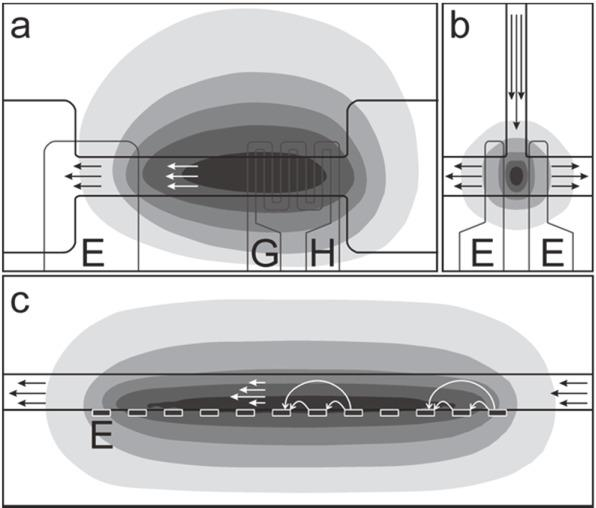Top- (a, b) and side-view (c) sketches of directly heated ETμP (a), T-channel ETμP (b), and ETTWμP (c) structures with temperature distributions in gray scaling (one gray step corresponds to approximately 1 K), fluid channel and metal-layer geometries. For the heat conductivities of the channel walls, bottom and cover see Stubbe et al. . Circumferences of field electrodes (E) and fluid channels are marked by thin and bold lines, respectively. G and H in (a) designate the common ground connector for field generation and the resistive heating meander. Straight arrows designate averaged medium velocities below the Maxwell-Wagner frequency (a and b) or in the β-dispersion range (c). Bent arrows in (c) sketch the effective AC and TW field distribution.

Pumping in Figure 12a is based on forces induced by a largely homogeneous electric field. The medium is asymmetrically heated with respect to the distribution of the electric field by Joule heating and a resistive heating element inside a channel of symmetric geometry. Heating forms a "smeared object" in the medium whose electric properties are characterized by a temperature-dependent increase in conductivity of approximately 2% and a permittivity decrease of approximately 0.4% per degree [Stubbe and Gimsa 2013]. As a result, the polarizability of the heated "smeared object" is increased below the Maxwell-Wagner frequency and decreased above this frequency when the ionic bulk conductivity is superseded by displacement currents. Below the Maxwell-Wagner frequency, the pump effect can intuitively be compared to the electrostatic pulling force acting on elder pith by the boundary-field effect of a plate capacitor. The "smeared object" is expelled from the field when the ionic bulk conductivity is superseded by displacement currents above the Maxwell-Wagner frequency resulting in a reversal of the pump direction [Stubbe et al. 2007]. In both cases, the system tends to replace the lower by the higher polarizable medium.

In Figure 12b, the "smeared object" is formed by Joule heating of the pumping field. Both the electric and the thermal fields are largely symmetrical with the same symmetry planes. A symmetry brake is introduced by the channel geometry. The pump effect can intuitively be explained by ED of the "object" in the homogenous field between the electrodes (compare to Figures 2, and 6a). The pump direction changes from "elongation" to "compression" around the Maxwell-Wagner frequency.

ETTWμPs (Figure 12c) exploit field-induced forces acting on charges which are induced in the bulk medium out-of-phase with respect to the field. Medium pumping (straight arrows) results from the superposition of all forces acting in the medium [Morgan et al. 2001, Ramos et al. 2005]. Charges and forces are induced most efficiently by field components which are oriented in the direction of the temperature gradient, i.e. perpendicular to the gray-step borders in Figure 12c. The white bent arrows sketch an instantaneous moment in the effective TW field distribution. For a clearer presentation, field components with opposite orientations are neglected. Close to the electrode plane, bent field lines bridge the gaps between neighbouring electrodes, i.e. AC components dominate the field. Travelling field components are more pronounced further away from the electrode plane (long bent arrows). Especially the interaction of these components with charges which are induced in the bulk out-of-phase with the field contributes to the overall pumping force. The situation is analogous to the field distribution in four-electrode ER chambers where strongly elliptical, i.e. largely linear AC fields are found in the vicinity of the electrodes, especially in between neighbouring electrodes. An ideally circular polarized field is found in the centre of the chamber [Maswiwat et al. 2006]. In analogy to the above considerations on ETμPs, the symmetry break in ETTWμPs arises from the directed TW-field movement.

While the bent arrows cross gray-steps that are almost oriented in pump direction in the central area of the pump channel, the gray-step borders are oriented in a more perpendicular way in the peripheral regions of the electrode array. Accordingly, pump-force contributions will be more effectively induced in the two peripheral areas.

Combined considerations of the electric and temperature field distributions suggest an increase in pump efficiency, e.g. due to external medium cooling in the center of the channel or alternatively, by a central gap in the electrode structure. Instead of one elongated "smeared object", two objects will be formed. Taken together, the two objects will exhibit more areas with force contributions that are aligned with the pump channel. The pumping efficiency can also be improved by externally induced longitudinal thermal gradients as already observed by Garcia-Sanchez et al. .

In Figure 12c, the temperature gradient runs from top to bottom in the central area of the electrode structure. When the pumping effect is dominated by force contributions generated in this area, the pump direction can be reversed by reversing the orientation of the gradient, e.g. by external heating or cooling [Liu et al. 2014].

An intuitive explanation for this effect can be obtained from a comparison of the field distributions in the center of Figure 12c with Figure 2. In a thought experiment, the bent field lines are straightened in order to allow for an easier comparison to the relations presented in Figure 2. If straightened, the long field line would pass through a virtual, smeared object of reduced temperature, starting and ending in pumping medium with an elevated temperature near the electrode plane, given the temperature distribution of Figure 12c. Qualitatively, this corresponds to Figure 2a, i.e. the smeared object is less polarizable than the surrounding medium when the conductivity polarization dominates. Even though the polarizability of the smeared object decreases in the dispersion range, its polarizability increases relative to that of the surrounding medium. In ER, an increasing effective polarizability of the object results in counter-field rotation (Figures 8a and 9). Accordingly, pumping in Figure 12c will be induced against the direction of movement of the TW field.

A reversal of the temperature gradient, for example by external heating or cooling, will inverse the above relations, that is, the smeared object will become more polarizable than the surrounding medium (Figure 1b). The pumping direction will be reversed accordingly. When a temperature gradient is externally imposed, perpendicular to the channel, the pump effect can be improved by an additional electrode array at the top of the pumping channel, inducing a field that travels in a direction opposite to the field at the bottom [Liu et al. 2014].

Because TW pumping relies on out-of-phase polarization, dispersion processes are a general prerequisite for pumping. In the β-dispersion range, these may be dispersions of the polarization of "smeared structural interfaces" in the pumping medium, but also a thick dielectric layer between the electrode plane and the aqueous pump medium can form a dispersive structural interface. Such a layer may improve TW pumping performance by improving the TW field distribution inside the pumping medium and can also be used to adjust the dispersion frequency [Marczak and Diesinger 2009]. The layer excludes pumping medium from the low-force range close to the electrode plane where the spatial TW field effect is ineffective, because AC components of the electrostatic field dominate. Also medium volumes at distances far from the electrode plane will not experience a TW force because the parallax between the single electrodes will result in a virtually equipotential electrode-array area [Morgan et al. 2001]. For a high pumping force, the pump-channel geometry should confine the electrothermal (electrostatic) TW effect to a certain distance range from the electrodes.

Nevertheless, in the frequency range below approximately 10 kHz, out-of-phase components are also generated by electric dispersions of the double-layer polarization (electric α-dispersions, i.e. "concentration polarization") or dispersions of microfluidic flow patterns (electroosmosis, "hydrodynamic α-dispersion") (for a discussion see Georgieva et al. 1998, Gimsa and Wachner 1998, Ramos et al. 2005, Zimmerman et al. 2002). In principle, these effects can be seen as the AC versions of DC electrokinetic effects and their frequency-dependent dispersion. Typically above 200 MHz, dipole molecules may also exhibit out-of-phase components due to Debye dispersions. The exploitation of Debye effects is not well investigated for pumping and will not be considered in this manuscript. Also DC-induced electro-osmotic flows which are known for a long time, will not be considered.

Nevertheless, because the majority of papers on TW pumping (or AC field pumping in asymmetrical electrode arrays) deal with electro-osmotic effects, a short note should be added in relation to the underlying mechanisms [Ajdari 2000, García-Sánchez et al. 2006, Morgan et al. 2001, Ramos et al. 2005, Urbanski et al. 2006]. In a number of papers, the qualitative differences of the pumping mechanisms that are based on α- or β-dispersion processes are not made clear or are even confused. One reason is that the (usually lower) α- and (usually higher) β-dispersion frequencies are not always well separated, depending on channel size and electric properties. While β-dispersions depend on the (largely constant) permittivity and the specific conductivity of the aqueous medium, α-dispersions depend on the (pH dependent) surface charge and ionic strength, i.e. the Debye-Hückel double-layer length [Maier 1997, Neu et al. 2002, Zimmerman et al. 2002]. Because ion concentration determines conductivity and ionic strength, it is the most important adjustable parameter which determines the frequencies of the α- and β-dispersions for a given system. Nevertheless, in the α-dispersion range, the different mechanisms involved are more complex than in the β-dispersion range discussed above.

Before the boom of microfluidic TW pumps, these relationships between the different α- and β-dispersion effects had been studied in ER experiments on single particles [Georgieva et al. 1998, Hughes et al. 1996, Maier 1997, Neu et al. 2002, Zimmerman et al. 2002]. Three α-effects, one electric and two electrokinetic effects influence the behaviour of a particle. First, electric polarization shifts the ion concentrations inside its electric double layer ("concentration polarization" with a time constant determined by the ionic diffusion coefficient and particle radius); second, the charged particle experiences electrophoretic movement in the external field (time-constant determined by particle mass and Stokes's friction); and last, and most complex, the electro-osmotic flow which is generated inside the double layer propagates hydrodynamically into the surrounding medium [García-Sánchez et al. 2006, Ramos et al. 2005]. To the best of our knowledge, these known relations have not yet been taken into account for the qualitative interpretation of TW pumping, even though the relations are simpler in pumps, because the "particle", i.e. the charged pump-channel wall, does not undergo electrophoretic movement.

Even though the electrokinetic and impedance properties of a system should be equivalent, this interrelation does not hold at the low frequencies in the α-dispersion range [Georgieva et al. 1998, Maier 1997, Neu et al. 2002, Zimmerman et al. 2002]. A qualitative explanation for this uncoupling is that the hydrodynamic time constants of the microsystems correspond to the α-dispersion-frequency range with frequencies typically below 1 kHz. In contrast, the field induced forces are time averaged over a field cycle for hydrodynamic reasons in the β-dispersion range, because the frequencies are too high to allow particle or medium movements to follow the alternations of the AC field.

Another reason for the more complex effects in the α-dispersion range is due to the fact that the forces originate from within the very thin electric double layers (typically <1 nm to <100 nm, depending on ionic strength). In a qualitative view, electro-osmotic TW pumping, e.g. by a square-topped four-phase field, is generated by the superposition of four electro-osmotic flow patterns [Morgan et al. 2001, Perch-Nielsen et al. 2004, Ramos et al. 2005]. The patterns, which are induced by the four consecutive electrode-drive patterns, sum up to the effective pumping effect. A similar mechanism induces electro-osmotic AC-field pumping in asymmetrical electrode arrays [Ajdari 2000, Urbanski et al. 2006].

While the actual forces are generated inside the very thin electric double layer in electro-osmotic pumps, forces are generated at the smeared structural interfaces inside the bulk medium of ETμPs or ETTWμPs (Figure 12) [Fuhr et al. 1992 and 1994, Morgan et al. 2001, Perch-Nielsen et al. 2004]. The two pump principles with their different localisations of force generation may overlap in an intermediate frequency range, causing a complex pumping behaviour [Garcia-Sanchez et al. 2009, Ramos et al. 2005]. For example, electrosomotic pump forces may induce pumping at the surface of the electrode array in one direction and the electrothermal TW effect may pump the bulk in the opposite direction.

For in-phase ETμPs, the pumping velocity vinp (Figures 12a) was shown to be:

$vinp=φηFEζ=−φεeΔTE21000η11−ωε0εeσe−121+ωε0εeσe−12$$${{v}_{inp}}=\frac{\varphi }{\eta }\left\langle {{F}_{E}} \right\rangle \zeta =-\frac{\varphi {{\varepsilon }_{e}}\Delta T{{E}^{2}}}{1000\eta }\left( \frac{11-{{\left( \omega {{\varepsilon }_{0}}{{\varepsilon }_{e}}\sigma _{e}^{-1} \right)}^{2}}}{1+{{\left( \omega {{\varepsilon }_{0}}{{\varepsilon }_{e}}\sigma _{e}^{-1} \right)}^{2}}} \right)$$

with $FE$$\left\langle {{F}_{E}} \right\rangle$being the volume-averaged pumping pressure given by the time-averaged volume force $FE$$\left\langle {{F}_{E}} \right\rangle$in the effective range of the pumping field ζ , i.e. in the pump channel volume [Stubbe et al. 2013]. ø , ε e , η , and ΔT are a specific channel-geometry coefficient, the permittivity of the medium, the medium viscosity, and the average temperature difference of the medium across distance ζ , respectively. Please note that φ does not only refer to the channel in the range of the pump structure itself but to the whole geometry contributing to viscous friction. The numbers 1000 and 11 result from the simplified characteristic temperature-dependent decrease of the permittivity by approximately 0.4% and the conductivity increase by approximately 2% per degree [Stubbe et al. 2013]. $1/ε0εeσe−1$$1/\left( {{\varepsilon }_{0}}{{\varepsilon }_{\text{e}}}\sigma _{\text{e}}^{-1} \right)$is the Maxwell-Wagner frequency, which is determined by the balance of the Ohmic and displacement currents (compare to equation (4)). For purposes of simplicity, it can assumed to be constant and temperature-independent. Equation 25 also applies to the structure of Figure 12b after φ and ΔT have been redefined.

While forces are generated by interaction with charges which are in-phase with the external field in DP and ETμPs, they are generated by the interaction with out-of-phase charges in ER and ETTWμP. The strong interrelations of the effects allows for the derivation of an expression for the pumping velocity voutp of ETTWμP by analogy considerations:

$voutp=φηFEζ=−φεeΔTE21000η12ωε0εeσe−11+ωε0εeσe−12$$${{v}_{outp}}=\frac{\varphi }{\eta }\left\langle {{F}_{E}} \right\rangle \zeta =-\frac{\varphi {{\varepsilon }_{e}}\Delta T{{E}^{2}}}{1000\eta }\left( \frac{12\omega {{\varepsilon }_{0}}{{\varepsilon }_{e}}\sigma _{e}^{-1}}{1+{{\left( \omega {{\varepsilon }_{0}}{{\varepsilon }_{e}}\sigma _{e}^{-1} \right)}^{2}}} \right)$$

(see also: Fuhr et al.  and , Gimsa et al. ). Nevertheless, as considered above, the force generation within a given cross-section of the pump channel in the electrode-array area will exhibit a stronger deviation and be less efficient than in ETμPs (see discussion above). Also ΔT is not well defined in equation 26 (compare to Figure 12c). In the equation, the resulting lower efficiency of the ETTWμP can be considered by a redefinition of factor φ .

Figure 13 presents the theoretical time-averaged volume forces for ETμP and ETTWμP given by equations 25 and 26. As in Figures 9 and 10, the relations of the in-phase (ETμP) and out-of-phase (ETTWμP) effects are related through the Kramers-Kronig relation. While the dielectric parameters of water result in a magnitude of the positive (conductivity) force plateau of ETμPs which is roughly 11 times that of the negative (permittivity) plateau, the force-peak of ETTWμPs around the Maxwell-Wagner dispersion frequency corresponds to one half of the distance between the ETμP plateaux. Neglecting the prefactor terms in equations 25 and 26, the frequency-dependent terms provide the integer numbers eleven and minus one for the ETμP plateaux and six (11+1)/2 for the ETTWμPs peak. Obviously, the ETTWμP-peak force cannot reach the conductivity-plateau force of ETμPs.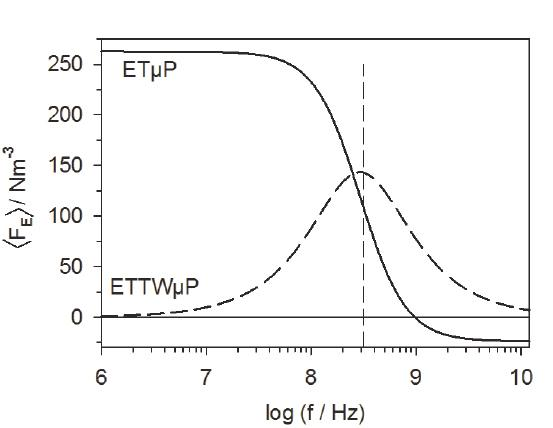Idealized time-averaged volume forces FE$\left\langle {{F}_{E}} \right\rangle$of aqueous medium with a relative permittivity of 80 and a specific conductivity of 1.3 S/m over field frequency. The solid curve corresponds to finite-element simulations for the ETμP structures of Figures 11a and 13b obtained for an effective field-electrode voltage of 20 VPP over 390 μm field-electrode distance and a temperature difference across the electrode gap of approximately 5 K [Stubbe et al. 2013]. The dashed line presents the maximum force generated in a ETTWμP under the same conditions. The pump-peak frequency corresponds to the center frequency between the two ETμP plateaus.

These considerations show that ETμPs outperform ETTWμP. ETTWμP need adjustment of the field frequency to the force peak, require more complex electrode structures and driving signals (see e.g.: Pethig et al. , Liu et al. , Marczak and Diesinger ). The maximum performance of ETTWμP is lower than that of ETμPs for physical reasons (peak vs. plateau heights) and it can only be reached with technological tricks, such as even more complex electrode structures (e.g. two TW-electrode arrays), additional dielectric layers, externally imposed temperature gradients, etc. Alternatively, the lower performance can be compensated for by higher temperature gradients. This is problematic, especially in biotech applications.

Examples of realized chip structures
DP collection of cells at the electrode pads of a multi-electrode array (MEA)

Multi-electrode arrays (MEAs) (Figure 14) are employed to detect the spontaneous electrical activity of cellular networks, e.g. of neuronal or myocardial cells in the investigation of the effects of chemical substances [Koester et al. 2010a]. The probability of cell-signal detection from a MEA is increased when the cell bodies adhere to the MEA electrode pads. DP collection can be applied to attract cells to the pads during cell seeding. Figure 14 shows a test experiment with baker's yeast cells seeded into a glass neurochip. The MEA was located in the centre of a circular 250 μl cell-culture trough that was glued to the chip. The cell-culture trough was filled with 200 μl of a 16 mS/m sucrose solution before cell-seeding. All MEA electrodes were electrically connected and energized by 16 Vrms, 2 MHz sinusoidal AC against a silver-wire ground electrode in the cell-culture trough.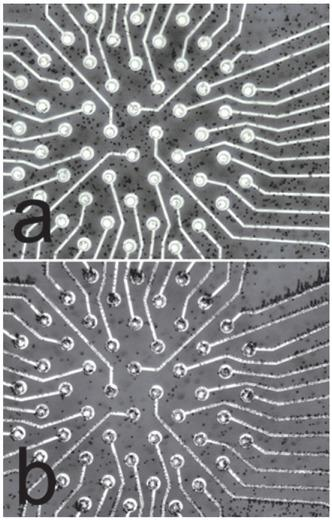DP collection of baker's yeast at the electrode pads of a glass neurochip with a 52 microelectrode MEA. The on-chip conducting paths of the MEA were 5 μm wide, insulated by >1μm silicon-nitride and terminated by circular pad openings with a diameter of 25 μm. The rectangular MEA-pad distances were 100 μm. All conducting structures were made from Pt. (a) Sedimented cells after cell seeding; (b) after 2 min of cell collection.

In an experiment with primary neuronal cells, cells were DP-allocated at the MEA pads and the DP medium was exchanged for cell-culture medium after cell adhesion approximately 2 hours after cell seeding. Neuronal networks exhibiting spontaneous electrical activity were formed after approximately 10 days of cell culture. Nevertheless, the method increased the success rate of action-potential detection from a certain MEA electrode only slightly (<10%).

ETμP structures

Figure 15 gives two examples for ETμP structures. For test measurements, the channel structures were made from photoresist and covered by a polydimethylsiloxane lid with two microfluidic ports for chamber filling. Aqueous medium was pumped in a closed, approximately 2 mm long fluidic loop. Pumping velocities were detected with microscopic tracer particles. For physiological solutions, they were in the order of 50 μms-1 at a temperature difference of approximately 5 K in a usable conductivity range of the pumping medium from extremely low to above physiological values [Stubbe 2007 and 2013]. Measurements of the pump-flow dependence on pump-medium properties, temperature gradient across the pump channel and on the frequency and strength of the driving AC-fields were consistent with finite-element simulations within an error range of approximately 10%. Additional inductances in the field electrode wiring allow for an increase of the pump velocity at low AC-field voltages in resonance conditions [Stubbe et al. 2013]. In principle, resonance effects can also be exploited in ETTWμPs.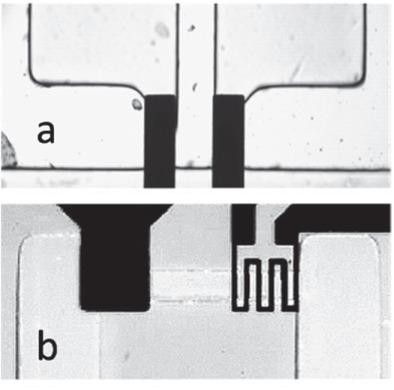Microscopic images of ETμP structures on thin glass according to Figures 12b and 12a (dark: 100 nm thick platinum structures; light gray: 60 μm thick polymer-wall structures; light areas: fluid channels). The central pump channels were 250 μm wide.

Advantages of ETμPs and ETTWμP over electrosmotic pumps are their high operational frequency ranging from kHz to GHz which avoids electrolytic processes and electrode deterioration. Optional passivation layers separate biological media and cells from the metallic surfaces and improve the protection of the platinum ETμP structures from electrolytic processes. Multiple ETμPs can be arranged in arrays in order to increase volume flow and/or pumping force. Depending on the pumping principle, pump channel-cross sections can be homogeneous, reducing the risk of channel clogging by debris.

Their properties predispose electrothermal pumps for the integration into LOCs for biological analysis, cell monitoring and cell culture systems. Even though ETTWμPs share many of the advantages of ETμPs, we believe that the latter will finally dominate LOC applications.

Metabolic cell chip with microfluidic structure

Chip-based cell-culture systems can be applied to reduce animal testing in the fields of medical diagnostics or drug development. In such LOCs, the amount of chemical test compounds required is reduced while simultaneously enabling easier parallelization of investigations [Buehler et al. 2011, Ehret et al. 1997, Shih et al. 2013, Ceriotti et al. 2007]. Up to now, only a few commercial systems have been available for the on-line monitoring of cell physiological parameters. For example, the Bionas® Discovery 2500 system (Bionas GmbH, Rostock, Germany) permits the non-invasive measurement of three metabolic parameters (pH, O2, and proliferation) [Ceriotti et al. 2007].

Figure 16 shows an example of a microfluidic multi-sensor chip. The chip has been developed for the monitoring of cell-physiological parameters and cell-proliferation behaviour. Glass-wafer technology ensured the microscopic observability of the on-chip cell culture. A mouse-osteoblast precursor-cell line (MC3T3-E1) was used as a model system (manuscript in preparation). The chip has been combined with a microfluidic channel grid, which was imprinted in poly-dimethyl-siloxane. The glass substrate bore thin-film platinum structures which were covered by 1 μm Si3N4 in most chip areas. Bare platinum structures were used for IDES for cell-proliferation monitoring and amperometric oxygen electrodes for the registration of respiration (oxygen consumption). For the chip in Figure 16, two on-chip ETμPs permitted a flow of medium to be induced throughout the micro-channel system and to circulate the solution in the fully closed system, e.g. for medium mixing and drug delivery. Two IDES types with 30-μm and 50-μm pitches were tested. Nevertheless, their sensitivity in the detection of cell-adhesion did not differ significantly. The oxygen sensors were circular Pt electrodes with a sensor area of approximately 100 μm2. Their sensitivity was 100 pA per 1% oxygen increase in the range from 0% to 21% oxygen. Medium acidification by cellular metabolism was monitored by potentiometric pH sensors which were covered with a thin (approximately 40 nm) pH-sensitive Si3N4 layer. The pH sensors showed a linear behaviour in the pH range from 4 to 9, with a sensitivity of up to 47 mV per pH step. During culture, the medium had to be exchanged periodically after 3 to 6 hours because of medium acidification by approximately one pH step within this time.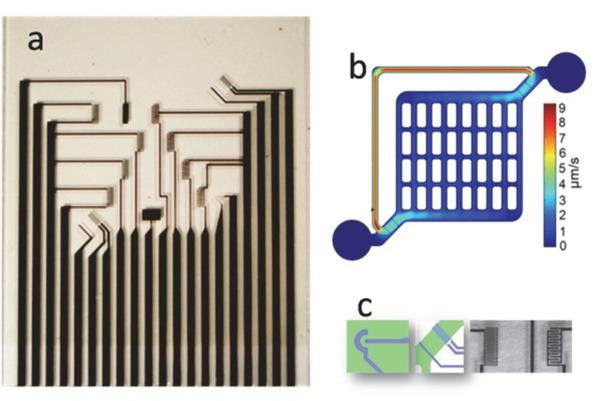(a) Glass cell-culture chip (size: half a microscopic slide) with two ETμPs according to Figure 12a (diagonally oriented structures at the lower left and upper right corners), eight IDES for impedance detection of cell adhesion (light rectangular structures), one oxygen sensor (fine structure with two connectors at the lower right) and two pH sensors (dark rectangles at the lower center and in the top row). (b) ETμP-flow simulation in the closed microfluidic poly-dimethyl-siloxane structure. The highest velocity is reached in the back-flow path. (c) All sensors and pump structures were located in the fluid channels. From the left: oxygen sensor, ETμP, two 1.4 x 0.5 mm2 IDES with 30 μm and 50 μm pitches.

Acidification rate, oxygen decrease and IDES impedance can be used to characterize metabolic cell activity and proliferation [Buehler et al. 2011]. In the setup, the cell culture could typically be maintained for up to 4 to 7 days. It was limited by gas-bubble formation in the narrow channels.

One clear advantage of the system is the bonus of sterile conditions in a simple incubator when the external medium-supply bottle is aerated through a sterile filter and an external peristaltic pump is located between the chip system and the waste bottle.

Integrated ETμPs allow the diffusion limits for the cellular supply with oxygen and nourishing molecules to be overcome, permit catabolites to be drawn out, and distribute chemical compounds to be tested in the cell culture. For this purpose, a widened flow-return path will provide a reservoir, which, in future systems, will be accessed through fluidic ports. For these, a clear advantage of integrated pumps is the ease of keeping the self-contained system sterile. While the integrated sensors allow for the real-time control of physiological parameters, micropumps permit the adjustment of these parameters according to the demands of the cell culture.

In the future, the number of applications of sensorized cell-culture systems is expected to rise for medical test systems and basic research on cell physiology [El-Ali et al. 2006]. Future chip designs may also feature modified sensors for other compounds, which may be based on direct optical, fluorescence or electric detection with potentiometric, amperometric, and impedance principles, as these are already in use [Shih et al. 2013, Kafka et al. 2008, Pan et al. 2004].Two complementary models consisting of blocks of air and water representing the membranous and aqueous media in cellular systems. a) Air block confined by two water blocks. b) Water block confined by air blocks. The external electrode charges influence charges in water but not in air. Induced charges are drawn for DC or AC for the half-wave during which the left electrode is positively charged.Complementary polarization and force effects in air-water models. Induced charges are located in the medium with the higher polarizability. The interaction of these charges with the electrode charges, i.e. the external field, leads to a compression of the air bubble (a) and an elongation of the water droplet (b).Single-shell cell model obtained by introducing the water droplet (Figure 2b) into a slightly larger air bubble (Figure 2a), leaving an air film (i.e. a "biological membrane") around the droplet’s surface. It was assumed that the external bulk medium has a lower conductivity than the internal bulk while both bulk media are isotonic.The (horizontally oriented) external field (component) Ea induces the potentials Ψ0a=zEa  and  Ψca*$\Psi _{0}^{a}=z{{E}_{a}}\,\,and\,\,\Psi _{c}^{a*}$in the absence (subscript 0) and the presence (subscript c) of an ellipsoidal (cellular) object at the site of the pole of its semiaxis a. Ψca*$\Psi _{c}^{a*}$is highest for a vacuum object with a polarizability comparable to that of a membrane covered cell at low frequency. For example, a vacuum sphere attracts the equipotential plane that is located at the "influential radius" ainf distance of 3a/ 2 from the symmetry plane, which can be assumed to be at 0 V. While the maximum of Ψca*=ainfEa$\Psi _{c}^{a*}={{a}_{\inf }}{{E}_{a}}$is determined by the influential radius, i.e. the geometry of the object, its actual value is determined by the effective electric properties of object and suspension medium.Equipotential plane distribution in and around a spherical single-shell model of radius r . The external distributions are identical for Maxwell's equivalent body (sketched for the left hemisphere) and the membrane-covered object (sketched for the right hemisphere). Important points in the model are marked by circles. The potentials at these points can be obtained from the RC-model at the bottom, which permits calculation of the induced dipole moment and transmembrane potential. From the existence of Maxwell's equivalent body, it follows that electric measurements (detecting the induced dipole moment) do not allow one to distinguish whether frequency-dependent object properties stem from internal structures or from the frequency-dependent properties of the media of which the object is composed.(a) ED according to Figure 2. (b) For three-axial, ellipsoidal objects each axis may be oriented in parallel to the field. Orientation depends on field frequency, object and medium properties. The field electrodes as well as the possible (frequency-dependent) forces and torques are marked by bold dark lines and arrows, respectively.(a) Evenly polarizable objects always attract one another (compare to charges in Figure 2) leading to the formation of pairs or pearl-chains. (b) Elongation and compression according to Figure 2a and b translate into positive or negative DP, i.e. movement of the object towards high and low field, respectively. (c) Strongly inhomogeneous fields can be used for the collection of submicro-objects or field caging.(a) Rotating fields may induce a torque leading to object rotation in (increasing effective polarizability of object with frequency) or against (decreasing effective polarizability) the direction of field rotation. (b) This torque corresponds to the translational forces in TWDP. Lifting or attracting forces correspond to the DP forces in Figure 7b.Real and imaginary parts of the Clausius-Mossotti factor (full lines, left ordinate) as well as the induced transmembrane potential (dashed line, right ordinate) at the pole of a spherical cell model (Table 1). Dotted lines: spectra are reduced to the membrane dispersion when the permittivities of the bulk media are neglected. Vertical dashed line: -3 dB frequency of the absolute value of the induced transmembrane potential.Real and imaginary parts of the specific impedance (IMP=1/σS*)$\left( \text{IMP=}{1}/{\sigma _{S}^{*}}\; \right)$of a cell suspension over frequency (top), as well as in the complex plane (bottom) calculated for the parameters of Table 1 (full lines, equation (18)). The spectra are reduced to the membrane dispersion when the permittivities of the bulk media are neglected (dotted lines, equation (19)). The characteristic membrane-dispersion frequency of the suspension fc1IMP$f_{c1}^{IMP}$corresponds to the frequency of the peak in the imaginary part. In the complex plot, it is the frequency of the point obtained by dropping a perpendicular from the center of the semicircle.Volume fraction dependencies of fc1IMP$f_{c1}^{IMP}$obtained from four different models for σi>>σe,   σi=σe,${{\sigma }_{i}}>>{{\sigma }_{e}},\,\,\,{{\sigma }_{i}}={{\sigma }_{e}},$and σi=0.001σe.${{\sigma }_{i}}=0.001{{\sigma }_{\text{e}}}.$Equation (18) corresponds to the complete model of Foster and Schwan  (dotted lines). While their simplified fc1IMP$f_{c1}^{IMP}$expression slightly increases with p (full lines), the new equation (20) predicts a moderate decrease (dashed lines), in accordance with the complete models. In all models, the points for p=0 are identical with fc1IMP=fc1$[f_{c1}^{IMP}={{f}_{c1}}$(equations 20-23).Top- (a, b) and side-view (c) sketches of directly heated ETμP (a), T-channel ETμP (b), and ETTWμP (c) structures with temperature distributions in gray scaling (one gray step corresponds to approximately 1 K), fluid channel and metal-layer geometries. For the heat conductivities of the channel walls, bottom and cover see Stubbe et al. . Circumferences of field electrodes (E) and fluid channels are marked by thin and bold lines, respectively. G and H in (a) designate the common ground connector for field generation and the resistive heating meander. Straight arrows designate averaged medium velocities below the Maxwell-Wagner frequency (a and b) or in the β-dispersion range (c). Bent arrows in (c) sketch the effective AC and TW field distribution.Idealized time-averaged volume forces FE$\left\langle {{F}_{E}} \right\rangle$of aqueous medium with a relative permittivity of 80 and a specific conductivity of 1.3 S/m over field frequency. The solid curve corresponds to finite-element simulations for the ETμP structures of Figures 11a and 13b obtained for an effective field-electrode voltage of 20 VPP over 390 μm field-electrode distance and a temperature difference across the electrode gap of approximately 5 K [Stubbe et al. 2013]. The dashed line presents the maximum force generated in a ETTWμP under the same conditions. The pump-peak frequency corresponds to the center frequency between the two ETμP plateaus.DP collection of baker's yeast at the electrode pads of a glass neurochip with a 52 microelectrode MEA. The on-chip conducting paths of the MEA were 5 μm wide, insulated by >1μm silicon-nitride and terminated by circular pad openings with a diameter of 25 μm. The rectangular MEA-pad distances were 100 μm. All conducting structures were made from Pt. (a) Sedimented cells after cell seeding; (b) after 2 min of cell collection.Microscopic images of ETμP structures on thin glass according to Figures 12b and 12a (dark: 100 nm thick platinum structures; light gray: 60 μm thick polymer-wall structures; light areas: fluid channels). The central pump channels were 250 μm wide.(a) Glass cell-culture chip (size: half a microscopic slide) with two ETμPs according to Figure 12a (diagonally oriented structures at the lower left and upper right corners), eight IDES for impedance detection of cell adhesion (light rectangular structures), one oxygen sensor (fine structure with two connectors at the lower right) and two pH sensors (dark rectangles at the lower center and in the top row). (b) ETμP-flow simulation in the closed microfluidic poly-dimethyl-siloxane structure. The highest velocity is reached in the back-flow path. (c) All sensors and pump structures were located in the fluid channels. From the left: oxygen sensor, ETμP, two 1.4 x 0.5 mm2 IDES with 30 μm and 50 μm pitches.

#### Standard parameters of the spherical cell model.

ParameterValue
Cell radius (r)5 μm
Membrane thickness (d)8 nm
Conductivities
external (σe)0.1 S/m
membrane (σm)10-6 S/m corresponding to 125 S/m2
internal (σi)0.01σe to σi>>σe
Relative permittivities ε
external ( e)80
membrane (ε) mε9.04 corresponding to 0.01 F/m2
internal ( i)50
Volume fraction (p)≤10% (not for single cell calculations)
External field strength for calculation of transmembrane potential133.33 kV/m

Ajdari A. Pumping liquids using asymmetric electrode arrays. Phys. Rev. E 2000, 61: 45-48. http://dx.doi.org/10.1103/PhysRevE.61.R4510.1103/PhysRevE.61.R45AjdariAPumping liquids using asymmetric electrode arraysPhys. Rev. E2000614548http://dx.doi.org/10.1103/PhysRevE.61.R45

Asami K, Hanai T, Koizumi N. Dielectric approach to suspensions of ellipsoidal particles covered with a shell in particular reference to biological cells. Jpn. J. Appl. Phys. 1980, 19: 359-365. http://dx.doi.org/10.1143/JJAP.19.35910.1143/JJAP.19.359AsamiKHanaiTKoizumiNDielectric approach to suspensions of ellipsoidal particles covered with a shell in particular reference to biological cellsJpn. J. Appl. Phys198019359365http://dx.doi.org/10.1143/JJAP.19.359

Barat D, Spencer D, Benazzi, G, Mowlem MC, Morgan H. Simultaneous high speed optical and impedance analysis of single particles with a microfluidic cytometer. Lab Chip 2012, 12: 118-126. http://dx.doi.org/10.1039/c1lc20785g10.1039/C1LC20785G22051732BaratDSpencerDBenazziGMowlemMCMorganHSimultaneous high speed optical and impedance analysis of single particles with a microfluidic cytometerLab Chip201212118126http://dx.doi.org/10.1039/c1lc20785g

Becker FF, Wang XB, Huang Y, Pethig R, Vykoukal J, Gascoyne PRC. Separation of human breast cancer cells from blood by differential dielectric affinity. Proc. Natl. Acad. Sci. USA. 1995, 92: 860–864. http://dx.doi.org/10.1073/pnas.92.3.86010.1073/pnas.92.3.860BeckerFFWangXBHuangYPethigRVykoukalJGascoynePRCSeparation of human breast cancer cells from blood by differential dielectric affinityProc. Natl. Acad. Sci. USA199592860864http://dx.doi.org/10.1073/pnas.92.3.860

Bousse L, Mcreynolds RJ, Kirk G, Dawes T, Lam P, Bemiss WR. Micromachined multichannel systems for the measurement of cellular-metabolism. Sens. Actuators B-Chemical 1994, 20: 145-150. http://dx.doi.org/10.1016/0925-4005(94)01196-610.1016/0925-4005(94)01196-6BousseLMcreynoldsRJKirkGDawesTLamPBemissWRMicromachined multichannel systems for the measurement of cellular-metabolismSens. Actuators B-Chemical199420145150http://dx.doi.org/10.1016/0925-4005(94)01196-6

Bousse L, Parce W. Applying silicon micromachining to cellular-metabolism. IEEE Engin. Med. Biol. Mag. 1994, 13: 396-401. http://dx.doi.org/10.1109/51.29401110.1109/51.294011BousseLParceWApplying silicon micromachining to cellular-metabolismIEEE Engin. Med. Biol. Mag199413396401http://dx.doi.org/10.1109/51.294011

Buehler SM, Stubbe M, Gimsa U, Baumann W, Gimsa J. A decrease of intracellular ATP is compensated by increased respiration and acidification at sub-lethal parathion concentrations in murine embryonic neuronal cells: measurements in metabolic cell-culture chips. Tox. Lett. 2011, 207: 182-190. http://dx.doi.org/10.1016/j.toxlet.2011.09.00510.1016/j.toxlet.2011.09.005BuehlerSMStubbeMGimsaUBaumannWGimsaJA decrease of intracellular ATP is compensated by increased respiration and acidification at sub-lethal parathion concentrations in murine embryonic neuronal cells: measurements in metabolic cell-culture chipsTox. Lett2011207182190http://dx.doi.org/10.1016/j.toxlet.2011.09.00521939746

Ceriotti L, Kob A, Drechsler S, Ponti J, Thedinga E, Colpo P, Ehret R. Online monitoring of BALB/3T3 metabolism and adhesion with multiparametric chip-based system. Anal. Biochem. 2007, 371: 92-104. http://dx.doi.org/10.1016/j.ab.2007.07.01410.1016/j.ab.2007.07.01417709091CeriottiLKobADrechslerSPontiJThedingaEColpoPEhretROnline monitoring of BALB/3T3 metabolism and adhesion with multiparametric chip-based systemAnal. Biochem200737192104http://dx.doi.org/10.1016/j.ab.2007.07.01417709091

Daridon A, Fascio V, Lichtenberg J, Wutrich R, Langen H, Verpoorte E, de Rooij NF. Multi-layer microfluidic glass chips for microanalytical applications. Fresenius J. Anal. Chem. 2001, 371: 261-269. http://dx.doi.org/10.1007/s00216010100410.1007/s00216010100411678200DaridonAFascioVLichtenbergJWutrichRLangenHVerpoorteEdeRooij NFMulti-layer microfluidic glass chips for microanalytical applicationsFresenius J. Anal. Chem2001371261269http://dx.doi.org/10.1007/s00216010100411678200

Dunlop J, Bowlby M, Peri R, Vasilyev D, Arias R. High-throughput electrophysiology: an emerging paradigm for ion-channel screening and physiology. Nat. Rev. Drug Discov. 2008, 7: 358-368. http://dx.doi.org/10.1038/nrd25521835691910.1038/nrd2552DunlopJBowlbyMPeriRVasilyevDAriasRHigh-throughput electrophysiology: an emerging paradigm for ion-channel screening and physiologyNat. Rev. Drug Discov20087358368http://dx.doi.org/10.1038/nrd255218356919Search in Google Scholar

Dürr M, Kentsch J, Müller T, Schnelle T, Stelzle M. Microdevices for manipulation and accumulation of micro- and nanoparticles by dielectrophoresis. Electrophoresis 2003, 24: 722–731. http://dx.doi.org/10.1002/elps.2003900871260174410.1002/elps.200390087DürrMKentschJMüllerTSchnelleTStelzleMMicrodevices for manipulation and accumulation of micro- and nanoparticles by dielectrophoresisElectrophoresis200324722731http://dx.doi.org/10.1002/elps.200390087Search in Google Scholar

Ehret R, Baumann W, Brischwein M, Schwinde A, Stegbauer K, Wolf B. Monitoring of cellular behaviour by impedance measurements on interdigitated electrode structures. Biosens. Bioelectron. 1997, 12: 29-41. http://dx.doi.org/10.1016/0956-5663(96)89087-7897605010.1016/0956-5663(96)89087-7EhretRBaumannWBrischweinMSchwindeAStegbauerKWolfBMonitoring of cellular behaviour by impedance measurements on interdigitated electrode structuresBiosens. Bioelectron1997122941http://dx.doi.org/10.1016/0956-5663(96)89087-7Search in Google Scholar

El-Ali J, Sorger PK, Jensen KF. Cells on chips. Nature 2006, 442: 403-411. http://dx.doi.org/10.1038/nature0506310.1038/nature05063El-AliJSorgerPKJensenKFCells on chipsNature2006442403411http://dx.doi.org/10.1038/nature05063

Fiedler S, Shirley SG, Schnelle T, Fuhr G. Dielectrophoretic sorting of particles and cells in a microsystem. Anal. Chem. 1998, 70: 1909-1915. http://dx.doi.org/10.1021/ac971063b10.1021/ac971063bFiedlerSShirleySGSchnelleTFuhrGDielectrophoretic sorting of particles and cells in a microsystemAnal. Chem19987019091915http://dx.doi.org/10.1021/ac971063b

Foster KR, Schwan HP. 1996, Dielectric properties of tissues. Handbook of biological effects of electromagnetic fields. Polk C, Postow E (Eds.) CRC Press Inc., Boca Raton, FL. 25-102.FosterKRSchwanHP.1996Dielectric properties of tissues. Handbook of biological effects of electromagnetic fieldsPolkCPostowEEdsCRC Press IncBoca Raton, FL25102Search in Google Scholar

Fricke H. Relation of the permittivity of biological cell suspensions to fractional cell volume. Nature 1953, 172: 731–732. http://dx.doi.org/10.1038/172731a010.1038/172731a0FrickeHRelation of the permittivity of biological cell suspensions to fractional cell volumeNature1953172731732http://dx.doi.org/10.1038/172731a0

Fuhr G, Hagedorn R, Müller T, Benecke W, Wagner B. Microfabricated electrohydrodynamic (EHD) pumps for liquids of higher conductivity. J. Microelectromech. Syst. 1992, 1: 141-146. http://dx.doi.org/10.1109/84.18639310.1109/84.186393FuhrGHagedornRMüllerTBeneckeWWagnerBMicrofabricated electrohydrodynamic (EHD) pumps for liquids of higher conductivityJ. Microelectromech. Syst19921141146http://dx.doi.org/10.1109/84.186393

Fuhr G, Schnelle T, Wagner B. Travelling wave driven microfabricated electrohydrodynamic pumps for liquids. J. Micromech. Microeng. 1994, 4: 217-226. http://dx.doi.org/10.1088/0960-1317/4/4/00710.1088/0960-1317/4/4/007FuhrGSchnelleTWagnerBTravelling wave driven microfabricated electrohydrodynamic pumps for liquidsJ. Micromech. Microeng19944217226http://dx.doi.org/10.1088/0960-1317/4/4/007

Fuhr G, Müller T, Glasser H, Gimsa J, Hofmann U, Wagner B. Handling and investigation of adherently growing cells and viruses of medical relevance in three-dimensional micro-structures. MEMS 97, 1997. Proceedings - IEEE the Tenth Annual International Workshop on Micro Electro Mechanical Systems. 344-349.FuhrGMüllerTGlasserHGimsaJHofmannUWagnerBHandling and investigation of adherently growing cells and viruses of medical relevance in three-dimensional micro-structures. MEMS 97, 1997Proceedings - IEEE the Tenth Annual International Workshop on Micro Electro Mechanical Systems34434910.1109/MEMSYS.1997.581851Search in Google Scholar

Fuhr GR, Reichle C. Living cells in opto-electrical cages. Trends Anal. Chem. 2000, 19: 402-409. http://dx.doi.org/10.1016/S0165-9936(00)00015-710.1016/S0165-9936(00)00015-7FuhrGRReichleCLiving cells in opto-electrical cagesTrends Anal. Chem200019402409http://dx.doi.org/10.1016/S0165-9936(00)00015-7

García-Sánchez P, Ramos A, Green NG, Morgan H. Experiments on AC electrokinetic pumping of liquids using arrays of microelectrodes. J. Phys. D: Appl. Phys. 2006, 47: 075501García-SánchezPRamosAGreenNGMorganHExperiments on AC electrokinetic pumping of liquids using arrays of microelectrodesJ. Phys. D: Appl. Phys20064707550110.1109/TDEI.2006.1657983Search in Google Scholar

Georgieva R, Neu B, Shilov VM, Knippel E, Budde A, Latza R, Donath E, Kiesewetter, Bäumler H. Low frequency electrorotation of fixed red blood cells. Biophys. J. 1998, 74: 2114-2120. http://dx.doi.org/10.1016/S0006-3495(98)77918-4954507010.1016/S0006-3495(98)77918-4GeorgievaRNeuBShilovVMKnippelEBuddeALatzaRDonathEKiesewetterBäumler HLow frequency electrorotation of fixed red blood cellsBiophys. J19987421142120http://dx.doi.org/10.1016/S0006-3495(98)77918-4Search in Google Scholar

Gimsa J. New light-scattering and field-trapping methods access the internal structure of submicron particles, like influenza viruses. Riu PJ, Rosell J, Bragos R, Casas O (Eds.) Electrical bio-impedance methods. Applications to medicine and biotechnology. New York: Ann. New York Acad. Sciences. 1999, 287-298.GimsaJNew light-scattering and field-trapping methods access the internal structure of submicron particles, like influenza virusesRiuPJRosellJBragosRCasasOEdsElectrical bio-impedance methods. Applications to medicine and biotechnologyNew YorkAnn. New York AcadSciences199928729810.1111/j.1749-6632.1999.tb09476.xSearch in Google Scholar

Gimsa J. A comprehensive approach to electro-orientation, electro-deformation, dielectrophoresis, and electrorotation of ellipsoidal particles and biological cells. Bioelectrochem. 2001, 54: 23-31. http://dx.doi.org/10.1016/S0302-4598(01)00106-410.1016/S0302-4598(01)00106-4GimsaJA comprehensive approach to electro-orientation, electro-deformation, dielectrophoresis, and electrorotation of ellipsoidal particles and biological cellsBioelectrochem2001542331http://dx.doi.org/10.1016/S0302-4598(01)00106-4

Gimsa J, Eppmann P, Prüger B. Introducing phase analysis light scattering for dielectric characterization: Measurement of traveling-wave pumping. Biophys. J. 1997, 73: 3309-3316. http://dx.doi.org/10.1016/S0006-3495(97)78355-310.1016/S0006-3495(97)78355-39414241GimsaJEppmannPPrügerBIntroducing phase analysis light scattering for dielectric characterization: Measurement of traveling-wave pumpingBiophys. J19977333093316http://dx.doi.org/10.1016/S0006-3495(97)78355-3

Gimsa J, Glaser R, Fuhr G. Theory and application of the rotation of biological cells in rotating electric fields (electrorotation). Schütt W, Klinkmann H, Lamprecht I, Wilson T (Eds.) Physical characterization of biological cells (Berlin: Verlag Gesundheit GmbH Berlin) 1991, 295-323.GimsaJGlaserRFuhrGTheory and application of the rotation of biological cells in rotating electric fields (electrorotation)SchüttWKlinkmannHLamprechtIWilsonTEdsPhysical characterization of biological cellsBerlinVerlag Gesundheit GmbH Berlin1991295323Search in Google Scholar

Gimsa J, Pritzen C, Donath E. Characterization of virus - red cell interaction by electrorotation. Stud. Biophys. 1989, 130: 123-131.GimsaJPritzenCDonathECharacterization of virus - red cell interaction by electrorotationStud. Biophys1989130123131Search in Google Scholar

Gimsa J, Wachner D. A unified RC-model for impedance, dielectrophoresis, electrorotation and induced transmembrane potential. Biophys. J. 1998, 75: 1107-1116. http://dx.doi.org/10.1016/S0006-3495(98)77600-310.1016/S0006-3495(98)77600-39675212GimsaJWachnerDA unified RC-model for impedance, dielectrophoresis, electrorotation and induced transmembrane potentialBiophys. J19987511071116http://dx.doi.org/10.1016/S0006-3495(98)77600-3

Gimsa J, Wachner D. A polarization model overcoming the geometric restrictions of Laplace's solution for spheroidal cells: Obtaining new equations for field induced forces and transmembrane potential. Biophys. J. 1999, 77: 1316-1326. http://dx.doi.org/10.1016/S0006-3495(99)76981-X1046574410.1016/S0006-3495(99)76981-XGimsaJWachnerDA polarization model overcoming the geometric restrictions of Laplace's solution for spheroidal cells: Obtaining new equations for field induced forces and transmembrane potentialBiophys. J19997713161326http://dx.doi.org/10.1016/S0006-3495(99)76981-XSearch in Google Scholar

Gimsa J, Wachner D. On the analytical description of transmembrane voltage induced on spheroidal cells with zero membrane conductance. Eur. Biophys. J. 2001, 30: 463-466. http://dx.doi.org/10.1007/s0024901001621171830110.1007/s002490100162GimsaJWachnerDOn the analytical description of transmembrane voltage induced on spheroidal cells with zero membrane conductanceEur. Biophys. J200130463466http://dx.doi.org/10.1007/s002490100162Search in Google Scholar

Glynne-Jones P, Hill M, Acoustofluidics 23: acoustic manipulation combined with other force fields. Lab Chip, 2013, 13: 1003-1010. http://dx.doi.org/10.1039/c3lc41369a10.1039/C3LC41369A23385298Glynne-JonesPHillMAcoustofluidics 23: acoustic manipulation combined with other force fieldsLab Chip20131310031010http://dx.doi.org/10.1039/c3lc41369a

Goater AD, Burt JPH, Pethig R. A combined travelling wave dielectrophoresis and electrorotation device: applied to the concentration and viability determination of Cryptosporidium. J. Phys. D: Appl. Phys. 1997, 30: L65–L69. http://dx.doi.org/10.1088/0022-3727/30/18/00110.1088/0022-3727/30/18/001GoaterADBurtJPHPethigRA combined travelling wave dielectrophoresis and electrorotation device: applied to the concentration and viability determination of CryptosporidiumJ. Phys. D: Appl. Phys199730L65L69http://dx.doi.org/10.1088/0022-3727/30/18/001

Griffin JL. Orientation of human and avian erythrocytes in radio-frequency fields. Exp. Cell Res. 1970, 61: 113-120. http://dx.doi.org/10.1016/0014-4827(70)90263-6543161010.1016/0014-4827(70)90263-6GriffinJLOrientation of human and avian erythrocytes in radio-frequency fieldsExp. Cell Res197061113120http://dx.doi.org/10.1016/0014-4827(70)90263-6Search in Google Scholar

Grom F, Kentsch J, Müller T, Schnelle T, Stelzle M. Accumulation and trapping of hepatitis A virus particles by electrohydrodynamic flow and dielectrophoresis. Electrophoresis 2006, 27: 1386 - 1393. http://dx.doi.org/10.1002/elps.20050041610.1002/elps.20050041616568408GromFKentschJMüller TSchnelleTStelzleMAccumulation and trapping of hepatitis A virus particles by electrohydrodynamic flow and dielectrophoresisElectrophoresis20062713861393http://dx.doi.org/10.1002/elps.200500416

Gross GW, Rhoades BK, Azzazy HME, Wu M-C. The use of neuronal networks on multielectrode arrays as biosensors, Biosens. Bioelectr. 1995, 10: 553–567. http://dx.doi.org/10.1016/0956-5663(95)96931-N10.1016/0956-5663(95)96931-NGrossGWRhoadesBKAzzazyHMEWuM-CThe use of neuronal networks on multielectrode arrays as biosensors, BiosensBioelectr199510553–567http://dx.doi.org/10.1016/0956-5663(95)96931-N

Guck J, Schinkinger S, Lincoln B, Wottawah F, Ebert S, Romeyke M, Lenz D, Erickson HM, Ananthakrishnan R, Mitchell D, Käs J, Ulvick S, Bilby C. Optical deformability as an inherent cell marker for testing malignant transformation and metastatic competence. Biophys. J. 2005, 88: 3689-3698. http://dx.doi.org/10.1529/biophysj.104.0454761572243310.1529/biophysj.104.045476GuckJSchinkingerSLincolnBWottawahFEbertSRomeykeMLenzDEricksonHMAnanthakrishnanRMitchellDKäs JUlvickSBilbyCOptical deformability as an inherent cell marker for testing malignant transformation and metastatic competenceBiophys. J20058836893698http://dx.doi.org/10.1529/biophysj.104.045476Search in Google Scholar

Hagedorn, R, Fuhr G, Müller T, Gimsa J. 1992. Traveling-wave dielectrophoresis of microparticles. Electrophoresis. 13: 49-54. http://dx.doi.org/10.1002/elps.1150130110158725410.1002/elps.1150130110HagedornRFuhrGMüllerTGimsaJ1992Traveling-wave dielectrophoresis of microparticlesElectrophoresis134954http://dx.doi.org/10.1002/elps.1150130110Search in Google Scholar

Haia A, Spira ME. On-chip electroporation, membrane repair dynamics and transient in-cell recordings by arrays of gold mushroom-shaped microelectrodes. Lab Chip, 2012, 12: 2865-2873. http://dx.doi.org/10.1039/c2lc40091j10.1039/c2lc40091j22678065HaiaASpiraMEOn-chip electroporation, membrane repair dynamics and transient in-cell recordings by arrays of gold mushroom-shaped microelectrodesLab Chip20121228652873http://dx.doi.org/10.1039/c2lc40091j

Hölzel R. Electrorotation of single yeast cells at frequencies between 100 Hz and 1.6 GHz. Biophys J. 1997, 73: 1103–1109. http://dx.doi.org/10.1016/S0006-3495(97)78142-6925182610.1016/S0006-3495(97)78142-6HölzelR.Electrorotation of single yeast cells at frequencies between 100 Hz and 1.6 GHzBiophys J19977311031109http://dx.doi.org/10.1016/S0006-3495(97)78142-6Search in Google Scholar

Hughes MP, Pethig R, Wang X-B Dielectrophoretic forces on particles in travelling electric fields. J. Phys. D: Appl. Phys. 1996, 29: 474-482. http://dx.doi.org/10.1088/0022-3727/29/2/02910.1088/0022-3727/29/2/029HughesMPPethigRWang X-B Dielectrophoretic forces on particles in travelling electric fields. JPhys. D: Appl. Phys199629474482http://dx.doi.org/10.1088/0022-3727/29/2/029

Jones TB. Electromechanics of Particles, Cambridge University Press, Cambridge, 1995. http://dx.doi.org/10.1017/CBO9780511574498JonesTBElectromechanics of ParticlesCambridge University PressCambridge1995http://dx.doi.org/10.1017/CBO978051157449810.1017/CBO9780511574498Search in Google Scholar

Kafka J, Pänke O, Abendroth B, Lisdat F. A label-free DNA sensor based on impedance spectroscopy. Electrochim. Acta. 2008, 53: 7467-7474. http://dx.doi.org/10.1016/j.electacta.2008.01.03110.1016/j.electacta.2008.01.031KafkaJPänkeOAbendrothBLisdatFA label-free DNA sensor based on impedance spectroscopyElectrochim. Acta20085374677474http://dx.doi.org/10.1016/j.electacta.2008.01.031

Koester PJ, Bühler SM, Stubbe M, Tautorat C, Niendorf M, Baumann W, Gimsa J. Modular glass chip system measuring the electric activity and adhesion of neuronal cells - application and drug testing with sodium valproic acid. Lab Chip 2010a, 10: 1579-1586. http://dx.doi.org/10.1039/b923687b10.1039/b923687bKoesterPJBühler SMStubbeMTautoratCNiendorfMBaumannWGimsaJModular glass chip system measuring the electric activity and adhesion of neuronal cells - application and drug testing with sodium valproic acidLab Chip2010a1015791586http://dx.doi.org/10.1039/b923687b20358045

Koester PJ, Tautorat C, Beikirch H, Gimsa J, Baumann W. Recording electric potentials from single adherent cells with 3D microelectrode arrays after local electroporation. Biosens. Bioelectr. 2010b, 26: 1731–1735. http://dx.doi.org/10.1016/j.bios.2010.08.00310.1016/j.bios.2010.08.003KoesterPJTautoratCBeikirchHGimsaJBaumannWRecording electric potentials from single adherent cells with 3D microelectrode arrays after local electroporationBiosens. Bioelectr2010b2617311735http://dx.doi.org/10.1016/j.bios.2010.08.003

Kovarik ML, Gach PC, Ornoff DM, Wang Y, Balowski J, Farrag L, Allbritton NL. Micro total analysis systems for cell biology and biochemical assays. Anal. Chem. 2012, 84: 516-540. http://dx.doi.org/10.1021/ac202611x10.1021/ac202611x21967743KovarikMLGachPCOrnoffDMWangYBalowskiJFarragLAllbrittonNLMicro total analysis systems for cell biology and biochemical assaysAnal. Chem201284516540http://dx.doi.org/10.1021/ac202611x

Laurell T, Petersson F, Nilsson A. Chip integrated strategies for acoustic separation and manipulation of cells and particles. Chem. Soc. Rev. 2007, 36: 492-506. http://dx.doi.org/10.1039/b601326k10.1039/B601326K17325788LaurellTPeterssonFNilssonAChip integrated strategies for acoustic separation and manipulation of cells and particlesChem. Soc. Rev200736492506http://dx.doi.org/10.1039/b601326k

Liu W, Ren Y, Shao J, Jiang H, Ding Y. A theoretical and numerical investigation of travelling wave induction microfluidic pumping in a temperature gradient. J. Phys. D: Appl. Phys. 2014, 47: 075501. http://dx.doi.org/10.1088/0022-3727/47/7/07550110.1088/0022-3727/47/7/075501LiuWRenYShaoJJiangHDingYA theoretical and numerical investigation of travelling wave induction microfluidic pumping in a temperature gradientJ. Phys. D: Appl. Phys201447075501http://dx.doi.org/10.1088/0022-3727/47/7/075501

Maier H. Electrorotation of colloidal particles and cells depends on surface charge. Biophys. J. 1997, 73: 1617-1626. http://dx.doi.org/10.1016/S0006-3495(97)78193-110.1016/S0006-3495(97)78193-19284328MaierHElectrorotation of colloidal particles and cells depends on surface chargeBiophys. J19977316171626http://dx.doi.org/10.1016/S0006-3495(97)78193-1

Marczak M, Diesinger H. Traveling wave dielectrophoresis micropump based on the dispersion of a capacitive electrode layer J. Appl. Phys. 2009, 105: 124511. http://dx.doi.org/10.1063/1.315278710.1063/1.3152787MarczakMDiesingerHTraveling wave dielectrophoresis micropump based on the dispersion of a capacitive electrode layer JAppl. Phys2009105124511http://dx.doi.org/10.1063/1.3152787

Marszalek P, Liu D-S, Tsong TY. Schwan equation and transmembrane potential induced by alternating electric field. Biophys. J. 1990, 58: 1053-1058. http://dx.doi.org/10.1016/S0006-3495(90)82447-410.1016/S0006-3495(90)82447-42248989MarszalekPLiuD-STsongTYSchwan equation and transmembrane potential induced by alternating electric fieldBiophys. J19905810531058http://dx.doi.org/10.1016/S0006-3495(90)82447-4

Maswiwat K, Holtappels M, Gimsa J. On the field distribution in electrorotation chambers - influence of electrode shape. Electrochim. Acta. 2006, 51: 5215-5220 http://dx.doi.org/10.1016/j.electacta.2006.03.04810.1016/j.electacta.2006.03.048MaswiwatKHoltappelsMGimsaJOn the field distribution in electrorotation chambers - influence of electrode shapeElectrochim. Acta20065152155220http://dx.doi.org/10.1016/j.electacta.2006.03.048

Morgan H, Izquierdo AG, Bakewell D, Green NG, Ramos A. The dielectrophoretic and travelling wave forces generated by interdigitated electrode arrays: analytical solution using Fourier series. J. Phys. D: App. Phys. 2001, 34: 1553-1561. http://dx.doi.org/10.1088/0022-3727/34/10/31610.1088/0022-3727/34/10/316MorganHIzquierdoAGBakewellDGreenNGRamosAThe dielectrophoretic and travelling wave forces generated by interdigitated electrode arrays: analytical solution using Fourier seriesJ. Phys. D: App. Phys20013415531561http://dx.doi.org/10.1088/0022-3727/34/10/316

Müller T, Gradl G, Howitz S, Shirley S, Schnelle T, G. Fuhr G. A 3-D microelectrode system for handling and caging single cells and particles. Biosens. Bioelec. 1999, 14: 247-256. http://dx.doi.org/10.1016/S0956-5663(99)00006-8MüllerTGradlGHowitzSShirleySSchnelleT, G.Fuhr GA 3-D microelectrode system for handling and caging single cells and particles. Biosens. Bioelec199914247256http://dx.doi.org/10.1016/S0956-5663(99)00006-810.1016/S0956-5663(99)00006-8Search in Google Scholar

Neu B, Georgieva R, Meiselman HJ, Bäumler H. Alpha- and beta-dispersion of fixed platelets: comparison with a structure-based theoretical approach. Coll. Surf. A: Physicochem. Eng. Aspects 2002, 197: 27-35. http://dx.doi.org/10.1016/S0927-7757(01)00860-310.1016/S0927-7757(01)00860-3NeuBGeorgievaRMeiselmanHJBäumlerH.Alpha- and beta-dispersion of fixed platelets: comparison with a structure-based theoretical approachColl. Surf. A: Physicochem. Eng. Aspects20021972735http://dx.doi.org/10.1016/S0927-7757(01)00860-3

Nilsson J, Evander M, Hammarström B, Laurell T. Review of cell and particle trapping in microfluidic systems. Anal. Chim. Acta 2009, 649: 141-157. http://dx.doi.org/10.1016/j.aca.2009.07.0171969939010.1016/j.aca.2009.07.017NilssonJEvanderMHammarströmBLaurellTReview of cell and particle trapping in microfluidic systemsAnal. Chim. Acta2009649141157http://dx.doi.org/10.1016/j.aca.2009.07.01719699390Search in Google Scholar

Oberti S, Neild A, Möller D, Dual J. Strategies for single particle manipulation using acoustic radiation forces and external tools. Phys. Procedia 2010, 3: 255-262. http://dx.doi.org/10.1016/j.phpro.2010.01.03410.1016/j.phpro.2010.01.034ObertiSNeildAMöller DDualJStrategies for single particle manipulation using acoustic radiation forces and external toolsPhys. Procedia20103255262http://dx.doi.org/10.1016/j.phpro.2010.01.034

Pan D, Chen J, Nie L, Tao W, Yao S. An amperometric glucose biosensor based on poly(o-aminophenol) and Prussian blue films at platinum electrode. Anal. Biochem. 2004, 324: 115-122. http://dx.doi.org/10.1016/j.ab.2003.09.02910.1016/j.ab.2003.09.02914654053PanDChenJNieLTaoWYaoSAn amperometric glucose biosensor based on poly(o-aminophenol) and Prussian blue films at platinum electrodeAnal. Biochem2004324115122http://dx.doi.org/10.1016/j.ab.2003.09.02914654053

Pauly H, Schwan HP. Über die Impedanz einer Suspension von kugelförmigen Teilchen mit einer Schale. Z. Naturforsch. 1959, 14b: 125-131. (in German)PaulyHSchwanHPÜber die Impedanz einer Suspension von kugelförmigen Teilchen mit einer Schale. ZNaturforsch195914b125131in German10.1515/znb-1959-0213Search in Google Scholar

Perch-Nielsen IR, Green NG, Wolff A. Numerical simulation of travelling wave induced electrothermal fluid flow. J. Phys. D: Appl. Phys. 2004, 37: 2323-2330.10.1088/0022-3727/37/16/016Perch-NielsenIRGreenNGWolffANumerical simulation of travelling wave induced electrothermal fluid flowJ. Phys. D: Appl. Phys20043723232330

Pethig R, Talary MS, Lee RS. Enhancing traveling-wave dielectrophoresis with signal superposition. IEEE Eng. Med. Biol. Mag. 2003, 22: 43-50. http://dx.doi.org/10.1109/MEMB.2003.12660461500799010.1109/MEMB.2003.1266046PethigRTalaryMSLeeRSEnhancing traveling-wave dielectrophoresis with signal superpositionIEEE Eng. Med. Biol. Mag2003224350http://dx.doi.org/10.1109/MEMB.2003.126604615007990Search in Google Scholar

Py C, Salim D, Monette R, Comas T, Fraser J, Martinez D, Martina M, Mealing G. Cell to aperture interaction in patch-clamp chips visualized by fluorescence microscopy and focused-ion beam sections. Biotech. Bioeng. 2011, 108: 1936-1941. http://dx.doi.org/10.1002/bit.2312710.1002/bit.23127PyCSalimDMonetteRComasTFraserJMartinezDMartinaMMealingGCell to aperture interaction in patch-clamp chips visualized by fluorescence microscopy and focused-ion beam sectionsBiotech. Bioeng201110819361941http://dx.doi.org/10.1002/bit.2312721391207

Ramos A, Morgan H, Green NG, González A, Castellanos A. Pumping of liquids with traveling-wave electroosmosis. J. Appl. Phys. 2005, 97: 084906. http://dx.doi.org/10.1063/1.187303410.1063/1.1873034RamosAMorganHGreenNGGonzálezACastellanosAPumping of liquids with traveling-wave electroosmosisJ. Appl. Phys200597084906http://dx.doi.org/10.1063/1.1873034

Retelj L, Pucihar G, Miklavcic D, Electroporation of intracellular liposomes using nanosecond electric pulses - a theoretical study. IEEE Trans. Biomed. Eng. 2013, 60: 2624–2635. http://dx.doi.org/10.1109/TBME.2013.2262177ReteljLPuciharGMiklavcicDElectroporation of intracellular liposomes using nanosecond electric pulses - a theoretical study. IEEE TransBiomed. Eng20136026242635http://dx.doi.org/10.1109/TBME.2013.226217710.1109/TBME.2013.226217723674414Search in Google Scholar

Schnelle T, Müller T, Reichle C, Fuhr G. Combined dielectrophoretic field cages and laser tweezers for electrorotation. Appl. Phys. B 2000, 70: 267-274. http://dx.doi.org/10.1007/s00340005004410.1007/s003400050044SchnelleTMüller TReichleCFuhrGCombined dielectrophoretic field cages and laser tweezers for electrorotationAppl. Phys. B200070267274http://dx.doi.org/10.1007/s003400050044

Schwan, HP. Biophysics of the interaction of electromagnetic energy with cells and membranes. In: Grandolfo M, Michaelson SM, Rindi A (Eds.) Biological effects and dosimetry of nonionizing radiation. 1983. Plenum Press, New York (USA), pp. 213-231. http://dx.doi.org/10.1007/978-1-4684-4253-3_9SchwanHPBiophysics of the interaction of electromagnetic energy with cells and membranesInGrandolfoMMichaelsonSMRindiAEdsBiological effects and dosimetry of nonionizing radiation1983Plenum PressNew York (USA)pp213231http://dx.doi.org/10.1007/978-1-4684-4253-3_910.1007/978-1-4684-4253-3_9Search in Google Scholar

Schwan HP, Schwarz G., Maczuk J, Pauly H. On the low-frequency dielectric dispersion of colloidal particles in electrolyte solution. J. Phys. Chem. 1962, 66: 2626-2635. http://dx.doi.org/10.1021/j100818a06610.1021/j100818a066SchwanHPSchwarzG.MaczukJPaulyHOn the low-frequency dielectric dispersion of colloidal particles in electrolyte solutionJ. Phys. Chem19626626262635http://dx.doi.org/10.1021/j100818a066

Schoenbach KH, Joshi RP, Kolb JF, Chen N, Stacey M, Blackmore PF, Buescher PF, Beebe SJ. Ultrashort electrical pulses open a new gateway into biological cells. Proc. IEEE 2004, 92: 1122-1137. http://dx.doi.org/10.1109/JPROC.2004.82900910.1109/JPROC.2004.829009SchoenbachKHJoshiRPKolbJFChenNStaceyMBlackmorePFBuescherPFBeebeSJUltrashort electrical pulses open a new gateway into biological cellsProc. IEEE20049211221137http://dx.doi.org/10.1109/JPROC.2004.829009

Shih SCC, Barbulovic-Nad I, Yang X, Fobel R, Wheeler AR. Digital microfluidics with impedance sensing for integrated cell culture and analysis. Biosens. Bioelectr. 2013, 42: 314-320. http://dx.doi.org/10.1016/j.bios.2012.10.03510.1016/j.bios.2012.10.035ShihSCCBarbulovic-NadIYangXFobelRWheelerARDigital microfluidics with impedance sensing for integrated cell culture and analysisBiosens. Bioelectr201342314320http://dx.doi.org/10.1016/j.bios.2012.10.035

Simeonova M, Wachner D, Gimsa J. Cellular absorption of electric field energy: influence of molecular properties of the cytoplasm. Bioelectrochem. 2002, 56: 215-218. http://dx.doi.org/10.1016/S1567-5394(02)00010-510.1016/S1567-5394(02)00010-5SimeonovaMWachnerDGimsaJCellular absorption of electric field energy: influence of molecular properties of the cytoplasmBioelectrochem200256215218http://dx.doi.org/10.1016/S1567-5394(02)00010-5

Stubbe M, Holtappels M, Gimsa J. A new working principle for ac electro-hydrodynamic on-chip micro-pumps. J. Phys. D: Appl. Phys. 2007, 40: 6850-6856. http://dx.doi.org/10.1088/0022-3727/40/21/05510.1088/0022-3727/40/21/055StubbeMHoltappelsMGimsaJA new working principle for ac electro-hydrodynamic on-chip micro-pumpsJ. Phys. D: Appl. Phys20074068506856http://dx.doi.org/10.1088/0022-3727/40/21/055

Stubbe M, Gyurova A, Gimsa J. Experimental verification of an equivalent circuit for the characterization of electrothermal micropumps: High pumping velocities induced by the external inductance at driving voltages below 5V. Electrophoresis 2013, 34: 562-574. http://dx.doi.org/10.1002/elps.20120034010.1002/elps.201200340StubbeMGyurovaAGimsaJExperimental verification of an equivalent circuit for the characterization of electrothermal micropumps: High pumping velocities induced by the external inductance at driving voltages below 5VElectrophoresis201334562574http://dx.doi.org/10.1002/elps.20120034023161729

Stubbe M, Gimsa, J. Electro-thermal Micro-pumps: exploiting structural polarizations at smeared interfaces. NSTI-Nanotech 2013, 2: 334-337.StubbeMGimsaJElectro-thermal Micro-pumps: exploiting structural polarizations at smeared interfacesNSTI-Nanotech20132334337Search in Google Scholar

Sun T, Morgan H. Single-cell microfluidic impedance cytometry: a review. Microfluid Nanofluid 2010, 8: 423-443. http://dx.doi.org/10.1007/s10404-010-0580-910.1007/s10404-010-0580-9SunTMorganHSingle-cell microfluidic impedance cytometry: a reviewMicrofluid Nanofluid20108423443http://dx.doi.org/10.1007/s10404-010-0580-9

Urbanski JP, Thorsen T, Levitan JA, Bazant MZ. Fast ac electro-osmotic micropumps with nonplanar electrodes. Appl. Phys. Lett. 2006, 89: 143508. http://dx.doi.org/10.1063/1.235882310.1063/1.2358823UrbanskiJPThorsenTLevitanJABazantMZFast ac electro-osmotic micropumps with nonplanar electrodesAppl. Phys. Lett200689143508http://dx.doi.org/10.1063/1.2358823

Wachner D, Simeonova M, Gimsa J. Estimating the subcellular absorption of electric field energy: equations for an ellipsoidal single shell model. Bioelectrochem. 2002, 56: 211-213. http://dx.doi.org/10.1016/S1567-5394(02)00020-810.1016/S1567-5394(02)00020-8WachnerDSimeonovaMGimsaJEstimating the subcellular absorption of electric field energy: equations for an ellipsoidal single shell modelBioelectrochem200256211213http://dx.doi.org/10.1016/S1567-5394(02)00020-8

Wolf B, Brischwein M, Grothe H, Stepper C, Ressler J, Weyh T. Lab-on-a-chip systems for cellular assays. In: Urban G (Ed.) BioMEMS. 2006. Springer, Dordrecht (NL), pp. 269-308.WolfBBrischweinMGrotheHStepperCResslerJWeyhTLab-on-a-chip systems for cellular assaysInUrbanGEdBioMEMS2006SpringerDordrecht (NL)pp26930810.1007/978-0-387-28732-4_9Search in Google Scholar

Yang CY, Lei U. Quasistatic force and torque on ellipsoidal particles under generalized dielectrophoresis. J. Appl. Phys. 2007, 102: 094702. http://dx.doi.org/10.1063/1.280218510.1063/1.2802185YangCYLeiUQuasistatic force and torque on ellipsoidal particles under generalized dielectrophoresisJ. Appl. Phys2007102094702http://dx.doi.org/10.1063/1.2802185

Zimmerman V, Shilov VN, López-Garcia JJ, Grosse C. Numerical calculation of the electrorotation velocity of latex-type particles. J. Phys. Chem. B 2002, 106: 13384-13392.10.1021/jp026127nZimmermanVShilovVNLópez-Garcia JJGrosseCNumerical calculation of the electrorotation velocity of latex-type particlesJ. Phys. Chem. B20021061338413392

• #### Body composition changes in the immediate peri-operative period following total joint arthroplasty

Recommended articles from Trend MD